• 当前卷积神经网络结构未能充分考虑RGB图像和深度图像独立性和相关性, 针对其联合检测效率不高问题, 提出了一种新双流卷积网络。将RGB图像和深度图像分别输入到两个卷积网络中, 两个卷积网络结构相同且权值共享...
• 针对低照度条件图像降质严重问题, 提出了一种基于深度卷积神经网络(DCNN)低照度图像增强算法。该算法根据Retinex模型合成训练样本, 将原始低照度图像从RGB (Red Green Blue)空间转换到HSI (Hue Saturation ...
• 针对低照度条件图像对比度不高、颜色失衡和存在噪声等问题,提出了一种基于多分支全卷积神经网络(MBACNN)低照度图像增强模型。该模型是一个端到端模型,包含特征提取模块(FEM)、增强模块(EM)、融合模块(FM)和...
• 根据鞋型识别独有特点,在DeepID基础上设计卷积神经网络框架,并构建鞋型样本数据库(50双鞋型样本,共计160231幅图像)。运用Caffe框架结合不同网络模型对鞋型图像数据进行训练和测试,实验设计初始网络结构由两层...
• ## TensorFlow 卷积神经网络之猫狗识别

万次阅读 多人点赞 2017-12-11 00:21:54
2. 设计神经网络 3. 进行训练测试 1. 数据处理 将图片数据处理为 tf 能够识别数据格式，并将数据设计批次。 第一步get_files() 方法读取图片，然后根据图片名，添加猫狗 label，然后再将 image和label 放到...
环境：python 3.6.1 :    Anaconda 4.4.0     tf 1.2.0
这份数据集来源于Kaggle，数据集有12500只猫和12500只狗。在这里简单介绍下整体思路
处理数据
设计神经网络
进行训练测试
1. 数据处理
将图片数据处理为 tf 能够识别的数据格式，并将数据设计批次。
第一步get_files() 方法读取图片，然后根据图片名，添加猫狗 label，然后再将 image和label 放到 数组中，打乱顺序返回
将第一步处理好的图片 和label 数组 转化为 tensorflow 能够识别的格式，然后将图片裁剪和补充进行标准化处理，分批次返回。
新建数据处理文件 ，文件名 input_data.py
import tensorflow as tf
import os
import numpy as np

def get_files(file_dir):
cats = []
label_cats = []
dogs = []
label_dogs = []
for file in os.listdir(file_dir):
name = file.split(sep='.')
if 'cat' in name:
cats.append(file_dir + file)
label_cats.append(0)
else:
if 'dog' in name:
dogs.append(file_dir + file)
label_dogs.append(1)
image_list = np.hstack((cats,dogs))
label_list = np.hstack((label_cats,label_dogs))
# print('There are %d cats\nThere are %d dogs' %(len(cats), len(dogs)))
# 多个种类分别的时候需要把多个种类放在一起，打乱顺序,这里不需要

# 把标签和图片都放倒一个 temp 中 然后打乱顺序，然后取出来
temp = np.array([image_list,label_list])
temp = temp.transpose()
# 打乱顺序
np.random.shuffle(temp)

# 取出第一个元素作为 image 第二个元素作为 label
image_list = list(temp[:,0])
label_list = list(temp[:,1])
label_list = [int(i) for i in label_list]
return image_list,label_list

# 测试 get_files
# imgs , label = get_files('/Users/yangyibo/GitWork/pythonLean/AI/猫狗识别/testImg/')
# for i in imgs:
# 	print("img:",i)

# for i in label:
# 	print('label:',i)
# 测试 get_files end

# image_W ,image_H 指定图片大小，batch_size 每批读取的个数 ，capacity队列中 最多容纳元素的个数
def get_batch(image,label,image_W,image_H,batch_size,capacity):
# 转换数据为 ts 能识别的格式
image = tf.cast(image,tf.string)
label = tf.cast(label, tf.int32)

# 将image 和 label 放倒队列里
input_queue = tf.train.slice_input_producer([image,label])
label = input_queue
# 读取图片的全部信息
image_contents = tf.read_file(input_queue)
# 把图片解码，channels ＝3 为彩色图片, r，g ，b  黑白图片为 1 ，也可以理解为图片的厚度
image = tf.image.decode_jpeg(image_contents,channels =3)
# 将图片以图片中心进行裁剪或者扩充为 指定的image_W，image_H
image = tf.image.resize_image_with_crop_or_pad(image, image_W, image_H)
# 对数据进行标准化,标准化，就是减去它的均值，除以他的方差
image = tf.image.per_image_standardization(image)

# 生成批次  num_threads 有多少个线程根据电脑配置设置  capacity 队列中 最多容纳图片的个数  tf.train.shuffle_batch 打乱顺序，
image_batch, label_batch = tf.train.batch([image, label],batch_size = batch_size, num_threads = 64, capacity = capacity)

# 重新定义下 label_batch 的形状
label_batch = tf.reshape(label_batch , [batch_size])
# 转化图片
image_batch = tf.cast(image_batch,tf.float32)
return  image_batch, label_batch

# test get_batch
# import matplotlib.pyplot as plt
# BATCH_SIZE = 2
# CAPACITY = 256
# IMG_W = 208
# IMG_H = 208

# train_dir = '/Users/yangyibo/GitWork/pythonLean/AI/猫狗识别/testImg/'

# image_list, label_list = get_files(train_dir)
# image_batch, label_batch = get_batch(image_list, label_list, IMG_W, IMG_H, BATCH_SIZE, CAPACITY)

# with tf.Session() as sess:
#    i = 0
#    #  Coordinator  和 start_queue_runners 监控 queue 的状态，不停的入队出队
#    coord = tf.train.Coordinator()
#    threads = tf.train.start_queue_runners(coord=coord)
#    # coord.should_stop() 返回 true 时也就是 数据读完了应该调用 coord.request_stop()
#    try:
#        while not coord.should_stop() and i<1:
#            # 测试一个步
#            img, label = sess.run([image_batch, label_batch])

#            for j in np.arange(BATCH_SIZE):
#                print('label: %d' %label[j])
#                # 因为是个4D 的数据所以第一个为 索引 其他的为冒号就行了
#                plt.imshow(img[j,:,:,:])
#                plt.show()
#            i+=1
#    # 队列中没有数据
#    except tf.errors.OutOfRangeError:
#        print('done!')
#    finally:
#        coord.request_stop()
#    coord.join(threads)
# sess.close()


2. 设计神经网络
利用卷积神经网路处理，网络结构为
# conv1   卷积层 1
# pooling1_lrn  池化层 1
# conv2  卷积层 2
# pooling2_lrn 池化层 2
# local3 全连接层 1
# local4 全连接层 2
# softmax 全连接层 3

新建神经网络文件 ，文件名 model.py
#coding=utf-8
import tensorflow as tf

def inference(images, batch_size, n_classes):

with tf.variable_scope('conv1') as scope:
# 卷积盒的为 3*3 的卷积盒，图片厚度是3，输出是16个featuremap
weights = tf.get_variable('weights',
shape=[3, 3, 3, 16],
dtype=tf.float32,
initializer=tf.truncated_normal_initializer(stddev=0.1, dtype=tf.float32))
biases = tf.get_variable('biases',
shape=,
dtype=tf.float32,
initializer=tf.constant_initializer(0.1))
conv = tf.nn.conv2d(images, weights, strides=[1, 1, 1, 1], padding='SAME')
pre_activation = tf.nn.bias_add(conv, biases)
conv1 = tf.nn.relu(pre_activation, name=scope.name)

with tf.variable_scope('pooling1_lrn') as scope:
pool1 = tf.nn.max_pool(conv1, ksize=[1, 3, 3, 1], strides=[1, 2, 2, 1], padding='SAME', name='pooling1')
norm1 = tf.nn.lrn(pool1, depth_radius=4, bias=1.0, alpha=0.001 / 9.0, beta=0.75, name='norm1')

with tf.variable_scope('conv2') as scope:
weights = tf.get_variable('weights',
shape=[3, 3, 16, 16],
dtype=tf.float32,
initializer=tf.truncated_normal_initializer(stddev=0.1, dtype=tf.float32))
biases = tf.get_variable('biases',
shape=,
dtype=tf.float32,
initializer=tf.constant_initializer(0.1))
conv = tf.nn.conv2d(norm1, weights, strides=[1, 1, 1, 1], padding='SAME')
pre_activation = tf.nn.bias_add(conv, biases)
conv2 = tf.nn.relu(pre_activation, name='conv2')

# pool2 and norm2
with tf.variable_scope('pooling2_lrn') as scope:
norm2 = tf.nn.lrn(conv2, depth_radius=4, bias=1.0, alpha=0.001 / 9.0, beta=0.75, name='norm2')
pool2 = tf.nn.max_pool(norm2, ksize=[1, 3, 3, 1], strides=[1, 1, 1, 1], padding='SAME', name='pooling2')

with tf.variable_scope('local3') as scope:
reshape = tf.reshape(pool2, shape=[batch_size, -1])
dim = reshape.get_shape().value
weights = tf.get_variable('weights',
shape=[dim, 128],
dtype=tf.float32,
initializer=tf.truncated_normal_initializer(stddev=0.005, dtype=tf.float32))
biases = tf.get_variable('biases',
shape=,
dtype=tf.float32,
initializer=tf.constant_initializer(0.1))
local3 = tf.nn.relu(tf.matmul(reshape, weights) + biases, name=scope.name)

# local4
with tf.variable_scope('local4') as scope:
weights = tf.get_variable('weights',
shape=[128, 128],
dtype=tf.float32,
initializer=tf.truncated_normal_initializer(stddev=0.005, dtype=tf.float32))
biases = tf.get_variable('biases',
shape=,
dtype=tf.float32,
initializer=tf.constant_initializer(0.1))
local4 = tf.nn.relu(tf.matmul(local3, weights) + biases, name='local4')

# softmax
with tf.variable_scope('softmax_linear') as scope:
weights = tf.get_variable('softmax_linear',
shape=[128, n_classes],
dtype=tf.float32,
initializer=tf.truncated_normal_initializer(stddev=0.005, dtype=tf.float32))
biases = tf.get_variable('biases',
shape=[n_classes],
dtype=tf.float32,
initializer=tf.constant_initializer(0.1))
softmax_linear = tf.add(tf.matmul(local4, weights), biases, name='softmax_linear')

return softmax_linear

def losses(logits, labels):
with tf.variable_scope('loss') as scope:
cross_entropy = tf.nn.sparse_softmax_cross_entropy_with_logits \
(logits=logits, labels=labels, name='xentropy_per_example')
loss = tf.reduce_mean(cross_entropy, name='loss')
tf.summary.scalar(scope.name + '/loss', loss)
return loss

def trainning(loss, learning_rate):
with tf.name_scope('optimizer'):
optimizer = tf.train.AdamOptimizer(learning_rate= learning_rate)
global_step = tf.Variable(0, name='global_step', trainable=False)
train_op = optimizer.minimize(loss, global_step= global_step)
return train_op

def evaluation(logits, labels):
with tf.variable_scope('accuracy') as scope:
correct = tf.nn.in_top_k(logits, labels, 1)
correct = tf.cast(correct, tf.float16)
accuracy = tf.reduce_mean(correct)
tf.summary.scalar(scope.name + '/accuracy', accuracy)
return accuracy

3. 训练数据，并将训练的模型存储
import os
import numpy as np
import tensorflow as tf
import input_data
import model

N_CLASSES = 2  # 2个输出神经元，［1，0］ 或者 ［0，1］猫和狗的概率
IMG_W = 208  # 重新定义图片的大小，图片如果过大则训练比较慢
IMG_H = 208
BATCH_SIZE = 32  #每批数据的大小
CAPACITY = 256
MAX_STEP = 15000 # 训练的步数，应当 >= 10000
learning_rate = 0.0001 # 学习率，建议刚开始的 learning_rate <= 0.0001

def run_training():

# 数据集
train_dir = '/Users/yangyibo/GitWork/pythonLean/AI/猫狗识别/img/'   #My dir--20170727-csq
#logs_train_dir 存放训练模型的过程的数据，在tensorboard 中查看
logs_train_dir = '/Users/yangyibo/GitWork/pythonLean/AI/猫狗识别/saveNet/'

# 获取图片和标签集
train, train_label = input_data.get_files(train_dir)
# 生成批次
train_batch, train_label_batch = input_data.get_batch(train,
train_label,
IMG_W,
IMG_H,
BATCH_SIZE,
CAPACITY)
# 进入模型
train_logits = model.inference(train_batch, BATCH_SIZE, N_CLASSES)
# 获取 loss
train_loss = model.losses(train_logits, train_label_batch)
# 训练
train_op = model.trainning(train_loss, learning_rate)
# 获取准确率
train__acc = model.evaluation(train_logits, train_label_batch)
# 合并 summary
summary_op = tf.summary.merge_all()
sess = tf.Session()
# 保存summary
train_writer = tf.summary.FileWriter(logs_train_dir, sess.graph)
saver = tf.train.Saver()

sess.run(tf.global_variables_initializer())
coord = tf.train.Coordinator()
threads = tf.train.start_queue_runners(sess=sess, coord=coord)

try:
for step in np.arange(MAX_STEP):
if coord.should_stop():
break
_, tra_loss, tra_acc = sess.run([train_op, train_loss, train__acc])

if step % 50 == 0:
print('Step %d, train loss = %.2f, train accuracy = %.2f%%' %(step, tra_loss, tra_acc*100.0))
summary_str = sess.run(summary_op)
train_writer.add_summary(summary_str, step)

if step % 2000 == 0 or (step + 1) == MAX_STEP:
# 每隔2000步保存一下模型，模型保存在 checkpoint_path 中
checkpoint_path = os.path.join(logs_train_dir, 'model.ckpt')
saver.save(sess, checkpoint_path, global_step=step)

except tf.errors.OutOfRangeError:
print('Done training -- epoch limit reached')
finally:
coord.request_stop()
coord.join(threads)
sess.close()

# train
run_training()

关于保存的模型怎么使用将在下一片博客中 展示。TensorFlow 卷积神经网络之使用训练好的模型识别猫狗图片
如果需要训练数据集可以评论留下联系方式。
原文完整代码地址：
https://github.com/527515025/My-TensorFlow-tutorials/tree/master/%E7%8C%AB%E7%8B%97%E8%AF%86%E5%88%AB
欢迎 star 欢迎提问。


展开全文• 将湍流影响下的高斯光束光斑图像作为神经网络输入,利用深度卷积神经网络提取图像特征信息,得到 Cn2大小,并采用平均绝对误差、平均相对误差、均方根方差和相关系数四个统计量来衡量模型估算效果。结果表明,该...
• 卷积神经网络应用：基于TensorflowCNN/CRF图像分割技术 【方向】 2016-12-26 14:27:03 浏览7815 评论0 Image TensorFlow 卷积神经网络 条件随机场 后处理 图像分割 上采样 摘要： 本篇文章...

卷积神经网络应用：基于Tensorflow的CNN/CRF图像分割技术

【方向】 2016-12-26 14:27:03 浏览7815 评论0

Image TensorFlow 卷积神经网络 条件随机场 后处理 图像分割 上采样

摘要： 本篇文章验证了卷积神经网络应用于图像分割领域时存在的一个问题——粗糙的分割结果。根据像素间交叉熵损失的定义，我们在简化的场景下进行了模型的训练，并使用后向传播来更新权重。我们使用条件随机场（CRFs）来解决分割结果粗糙的问题，并取得了很好的效果。本文中的代码注释详细、功能完善，也便于读者阅读。

这是一篇翻译文章。介绍了一种基于最近发布的TF-Slim库与预训练模型来进行图像分割的方法。本篇文章的内容包括基于条件随机场的模型训练与后处理过程。

引言

在之前的文章中，我们实现了上采样操作，并通过将其与scikit-image库中的对应实现作比较，以确保上采样过程的正确性。更具体地说，我们实现了论文《Fully convolutional networks for semantic segmentation》中描述的FCN-32分割网络。

在本篇文章中，我们将进行一个简单的训练：我们将从PASCAL VOC数据集中选取一张样本图像以及它的标注信息，基于样本图像与标注训练来我们的网络，并在同样的图像上测试我们训练好的网络。之所以这样做，是因为这样我们就可以使用CPU来训练模型——只需要10次迭代就能完成训练。本篇文章的另一个目的在于，证明我们这个网络（FCN-32s）的分割结果是很粗糙的——即使在用于训练的图像上进行分割，结果也同样如此。在本篇文章中，我们使用条件随机场的后处理阶段来解决这个问题，其综合考虑图像中的RGB纯色特征以及模型输出的预测概率，进而改善图像的分割效果。总的来说，我们得到了改善后的分割结果。我们刻意在文章中使用了非常简单的训练环境。类似的分割方法在Chen等人发表的论文《Semantic
Image Segmentation with Deep Convolutional Nets and Fully Connected CRFs》中也有所提及。请注意，本篇文章中的训练环境仅为了说明FCN-32s模型的局限，如果要在真实场景中进行训练，我们建议读者参考这篇论文《Fully convolutional networks for semantic segmentation》。

本篇文章使用Jupyter Notebook创建。在每个代码块后，你可以看到模型的评估结果。你也可以从这里获得本文对应的笔记文档。本篇文章的部分内容也借鉴了TF-Slim库的演示教程。

准备阶段

为了运行下面的代码，你需要安装Tensorflow。我使用的版本是r0.12。你可能需要使用Tensorflow的这个分支。

在这篇教程中，我也使用了scikit-imag库、numpy以及其他的一些依赖。你可以通过下载Anaconda的Python软件包来安装这些软件。

此外，你还需要按照之前几篇文章介绍的步骤来配置你的训练环境——包括如何下载VGG-16模型以及其他所有的必需操作。

上采样辅助函数与图像加载

在这一部分中，我们定义前一篇文章使用的辅助函数。如果你还记得的话，我们使用了上采样技术对我们从网络中获得的下采样预测结果进行采样。我们从VGG-16模型使用的最大值池化层中获得模型的下采样预测结果。

我们也为图像与各个实际分割区域的加载操作编写了程序。这些代码加入了足够的注释，所以不必担心无法理解它。
import numpy as np

def get_kernel_size(factor):
"""
给定所需的上采样因子，确定卷积核的大小
"""
return 2 * factor - factor % 2

def upsample_filt(size):
"""
创建一个给定(h, w) 大小的适用于上采样过程的二维双线性卷积核
"""
factor = (size + 1) // 2
if size % 2 == 1:
center = factor - 1
else:
center = factor - 0.5
og = np.ogrid[:size, :size]
return (1 - abs(og - center) / factor) * \
(1 - abs(og - center) / factor)

def bilinear_upsample_weights(factor, number_of_classes):
"""
使用双线性卷积核，为转置卷积创建权重矩阵
初始化
"""

filter_size = get_kernel_size(factor)

weights = np.zeros((filter_size,
filter_size,
number_of_classes,
number_of_classes), dtype=np.float32)

upsample_kernel = upsample_filt(filter_size)

for i in xrange(number_of_classes):

weights[:, :, i, i] = upsample_kernel

return weights

%matplotlib inline

from __future__ import division

import os
import sys
import tensorflow as tf
import skimage.io as io
import numpy as np

os.environ["CUDA_VISIBLE_DEVICES"] = '1'
sys.path.append("/home/dpakhom1/workspace/my_models/slim/")
checkpoints_dir = '/home/dpakhom1/checkpoints'

image_filename = 'cat.jpg'
annotation_filename = 'cat_annotation.png'

image_filename_placeholder = tf.placeholder(tf.string)
annotation_filename_placeholder = tf.placeholder(tf.string)
is_training_placeholder = tf.placeholder(tf.bool)

feed_dict_to_use = {image_filename_placeholder: image_filename,
annotation_filename_placeholder: annotation_filename,
is_training_placeholder: True}

image_tensor = tf.read_file(image_filename_placeholder)
annotation_tensor = tf.read_file(annotation_filename_placeholder)

image_tensor = tf.image.decode_jpeg(image_tensor, channels=3)
annotation_tensor = tf.image.decode_png(annotation_tensor, channels=1)

# 对于每个类别，将其设置为1而不是一个数字——我们在后续计算
# 交叉熵时会用到。有的时候，实际分割区域的掩码可以有很多值，
# 不仅仅为0和1
class_labels_tensor = tf.equal(annotation_tensor, 1)
background_labels_tensor = tf.not_equal(annotation_tensor, 1)

#将布尔值转换为浮点数——这样才能正确地计算交叉熵损失
bit_mask_class = tf.to_float(class_labels_tensor)
bit_mask_background = tf.to_float(background_labels_tensor)

combined_mask = tf.concat(concat_dim=2, values=[bit_mask_class,
bit_mask_background])

# 调整输入数据的大小，使其与tf.softmax_cross_entropy_with_logits中
# [batch_size, num_classes]的要求保持一致
flat_labels = tf.reshape(tensor=combined_mask, shape=(-1, 2))


损失函数定义与基于Adam优化算法的训练

在这一部分中，我们把准备好的所有东西整合到一起：为我们的网络添加上采样层，定义可微分求导的损失函数，并进行模型的训练。

参照论文《Fully convolutional networks for semantic segmentation》，我们将模型的损失定义为像素间的交叉熵。我们之所以这样定义，是因为在上采样后我们可以得到与输入图像尺寸相同的预测结果，进而我们可以将获得的分割结果与实际的各个分割区域进行比较：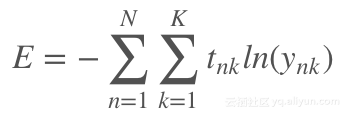其中，N表示像素的数量，K表示类别的数量，变量Tnk表示第n个像素对应的实际分割区域，实际区域以1至K的数字表示，变量Ynk为我们模型的预测结果（使用softmax函数来规范输出）。

对于这种情况，由于Adam优化器仅调整较少的参数就能使模型取得很好的效果，我们将其应用到了模型的训练当中。

在这个特殊的情况下，我们使用一张图像来训练模型并评估模型的效果——与真实场景相比，这种训练方式非常简单。我们这样做是为了展示这种方法的不足——只为了说明这种方法糟糕的定位能力。如果在这种简单场景下这种方法的效果尚且如此，那么在未训练的图像上，这种方法只会产生类似的更糟糕的结果。
import numpy as np
import tensorflow as tf
import sys
import os
from matplotlib import pyplot as plt

fig_size = [15, 4]
plt.rcParams["figure.figsize"] = fig_size

import urllib2

slim = tf.contrib.slim

from nets import vgg
from preprocessing import vgg_preprocessing

# 加载像素均值以及为每个像素进行减法运算的函数
from preprocessing.vgg_preprocessing import (_mean_image_subtraction,
_R_MEAN, _G_MEAN, _B_MEAN)

upsample_factor = 32
number_of_classes = 2
log_folder = '/home/dpakhom1/tf_projects/segmentation/log_folder'

vgg_checkpoint_path = os.path.join(checkpoints_dir, 'vgg_16.ckpt')

# 在与像素均值做差前，将图像转换至float32类型
image_float = tf.to_float(image_tensor, name='ToFloat')

# 将每个像素的具体数值与像素均值做差
mean_centered_image = _mean_image_subtraction(image_float,
[_R_MEAN, _G_MEAN, _B_MEAN])

processed_images = tf.expand_dims(mean_centered_image, 0)

upsample_filter_np = bilinear_upsample_weights(upsample_factor,
number_of_classes)

upsample_filter_tensor = tf.constant(upsample_filter_np)

# 定义将要使用的模型——指定在最后一层仅使用两个类别
with slim.arg_scope(vgg.vgg_arg_scope()):

logits, end_points = vgg.vgg_16(processed_images,
num_classes=2,
is_training=is_training_placeholder,
spatial_squeeze=False,
fc_conv_padding='SAME')

downsampled_logits_shape = tf.shape(logits)

# 计算上采样数据的输出大小
upsampled_logits_shape = tf.pack([
downsampled_logits_shape,
downsampled_logits_shape * upsample_factor,
downsampled_logits_shape * upsample_factor,
downsampled_logits_shape
])

# 进行上采样处理
upsampled_logits = tf.nn.conv2d_transpose(logits, upsample_filter_tensor,
output_shape=upsampled_logits_shape,
strides=[1, upsample_factor, upsample_factor, 1])

# 展开预测结果，以便于我们计算每个像素的交叉熵，并获得交叉熵的总和
flat_logits = tf.reshape(tensor=upsampled_logits, shape=(-1, number_of_classes))

cross_entropies = tf.nn.softmax_cross_entropy_with_logits(logits=flat_logits,
labels=flat_labels)

cross_entropy_sum = tf.reduce_sum(cross_entropies)

# 获得每个像素的最终预测结果——请注意，在这种情况下我们并不需要
# 使用softmax，因为我们只需要得到最终的决策。如果我们还需要各
# 个类别的概率，那么我们必须应用softmax
pred = tf.argmax(upsampled_logits, dimension=3)

probabilities = tf.nn.softmax(upsampled_logits)

# 在这里我们定义了一个优化器，并添加了所有将要创建至命名
# 空间'adam_vars'下的变量。这样做有利于我们后续轻松地访问
# 它们。这些变量供adam优化器使用，并且与vgg模型中的变量无关

# 我们还获得了每个变量的梯度数据
# 这样，我们可以在tensorboard中可视化这些变量
# optimizer.compute_gradients与optimizer.apply_gradients
# 等价于执行：
# train_step = tf.train.AdamOptimizer(learning_rate=0.0001).minimize(cross_entropy_sum)
with tf.variable_scope("adam_vars"):
optimizer = tf.train.AdamOptimizer(learning_rate=0.0001)
gradients = optimizer.compute_gradients(loss=cross_entropy_sum)

for grad_var_pair in gradients:

current_variable = grad_var_pair
current_gradient = grad_var_pair

# 替换原始变量名中的一些字符
# tensorboard不支持':'符号
gradient_name_to_save = current_variable.name.replace(":", "_")

# 得到每一层的梯度直方图，并随后在tensorboard中可视化这些数据
tensorboard
tf.summary.histogram(gradient_name_to_save, current_gradient)

train_step = optimizer.apply_gradients(grads_and_vars=gradients)

# 在这里，我们定义了一个函数，调用时会从VGG模型检查点中读取权重数据，并加载至变量中。
# 我们从负责类别预测的最后一层中剔除了权重。我们这样做是因为我们将有不同数量的
# 类别进行预测，我们不能在初始化时使用原先的类别。
vgg_except_fc8_weights = slim.get_variables_to_restore(exclude=['vgg_16/fc8', 'adam_vars'])

# 这里我们得到了网络中最后一层的权重变量
# 正如我们看到的，VGG最初训练的类别数量与我们实际的类别数量
# 并不相同——在我们的情况下，总共只有两类
vgg_fc8_weights = slim.get_variables_to_restore(include=['vgg_16/fc8'])

adam_optimizer_variables = slim.get_variables_to_restore(include=['adam_vars'])

# 为模型损失添加一个summary OP——以便我们可以在tensorboard中看到它
tf.summary.scalar('cross_entropy_loss', cross_entropy_sum)

# 将所有summary OP合并至一个OP总
# 在运行程序时生成字符串
merged_summary_op = tf.summary.merge_all()

# 创建一个summary writer——用于将所有日志写入到一个特定文件中
# 这个文件后续可以由tensorboard读取
summary_string_writer = tf.summary.FileWriter(log_folder)

# 如果日志文件夹尚未存在，则创建一个新的文件夹
if not os.path.exists(log_folder):
os.makedirs(log_folder)

# 创建一个OP，对VGG模型中各权重变量进行初始化操作
read_vgg_weights_except_fc8_func = slim.assign_from_checkpoint_fn(
vgg_checkpoint_path,
vgg_except_fc8_weights)

# 针对新的fc8层权重数据的初始化器——仅包括两类
vgg_fc8_weights_initializer = tf.variables_initializer(vgg_fc8_weights)

# adam变量的初始化器
optimization_variables_initializer = tf.variables_initializer(adam_optimizer_variables)

with tf.Session() as sess:
# 运行初始化器
read_vgg_weights_except_fc8_func(sess)
sess.run(vgg_fc8_weights_initializer)
sess.run(optimization_variables_initializer)

train_image, train_annotation = sess.run([image_tensor, annotation_tensor],
feed_dict=feed_dict_to_use)

f, (ax1, ax2) = plt.subplots(1, 2, sharey=True)
ax1.imshow(train_image)
ax1.set_title('Input image')
probability_graph = ax2.imshow(np.dstack((train_annotation,)*3)*100)
ax2.set_title('Input Ground-Truth Annotation')
plt.show()

# 执行10次迭代
for i in range(10):

loss, summary_string = sess.run([cross_entropy_sum, merged_summary_op],
feed_dict=feed_dict_to_use)

sess.run(train_step, feed_dict=feed_dict_to_use)

pred_np, probabilities_np = sess.run([pred, probabilities],
feed_dict=feed_dict_to_use)

summary_string_writer.add_summary(summary_string, i)

cmap = plt.get_cmap('bwr')

f, (ax1, ax2) = plt.subplots(1, 2, sharey=True)
ax1.imshow(np.uint8(pred_np.squeeze() != 1), vmax=1.5, vmin=-0.4, cmap=cmap)
ax1.set_title('Argmax. Iteration # ' + str(i))
probability_graph = ax2.imshow(probabilities_np.squeeze()[:, :, 0])
ax2.set_title('Probability of the Class. Iteration # ' + str(i))

plt.colorbar(probability_graph)
plt.show()

print("Current Loss: " +  str(loss))

feed_dict_to_use[is_training_placeholder] = False

final_predictions, final_probabilities, final_loss = sess.run([pred,
probabilities,
cross_entropy_sum],
feed_dict=feed_dict_to_use)

f, (ax1, ax2) = plt.subplots(1, 2, sharey=True)

ax1.imshow(np.uint8(final_predictions.squeeze() != 1),
vmax=1.5,
vmin=-0.4,
cmap=cmap)

ax1.set_title('Final Argmax')

probability_graph = ax2.imshow(final_probabilities.squeeze()[:, :, 0])
ax2.set_title('Final Probability of the Class')
plt.colorbar(probability_graph)

plt.show()

print("Final Loss: " +  str(final_loss))

summary_string_writer.close()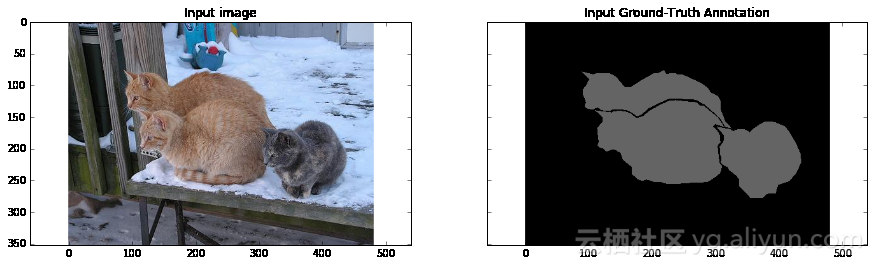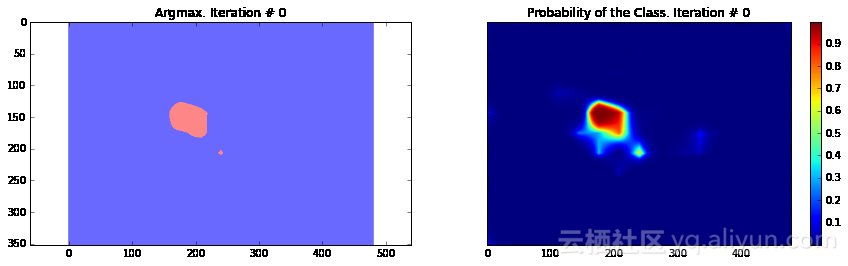Current Loss: 201433.0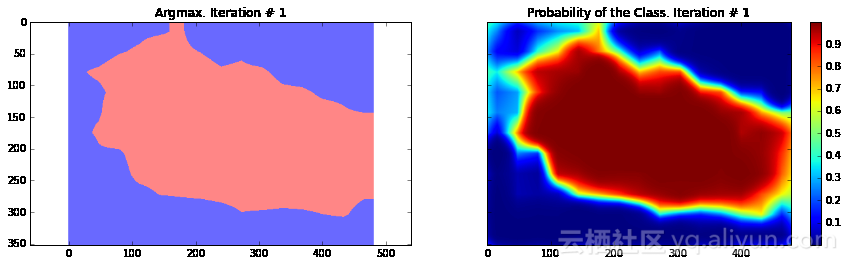Current Loss: 245565.0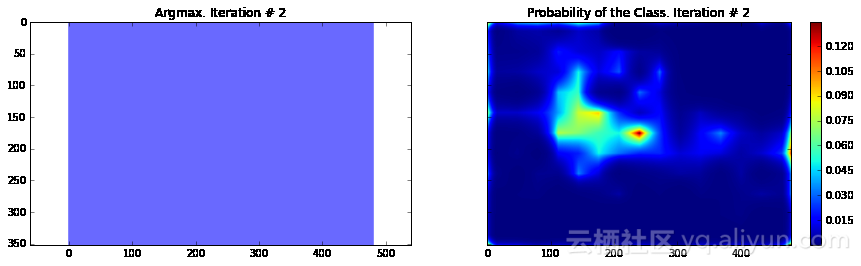Current Loss: 135906.0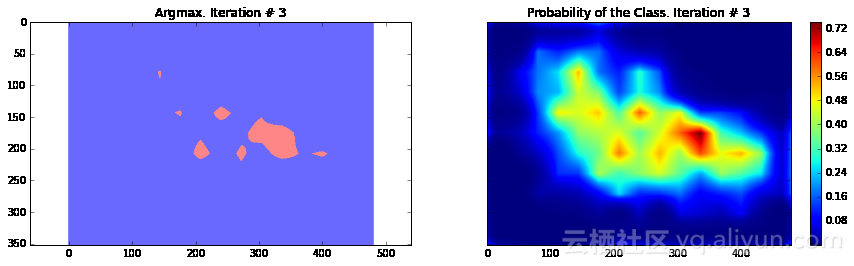Current Loss: 183353.0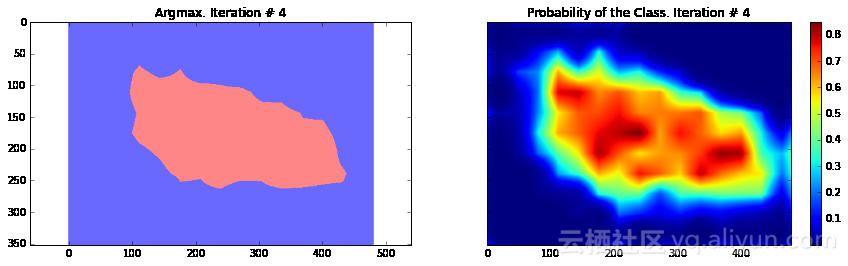Current Loss: 48563.9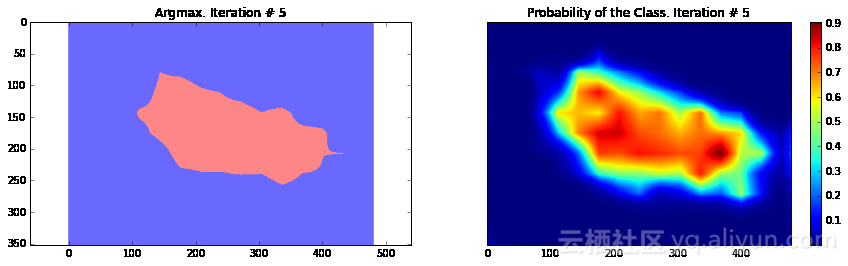Current Loss: 37925.8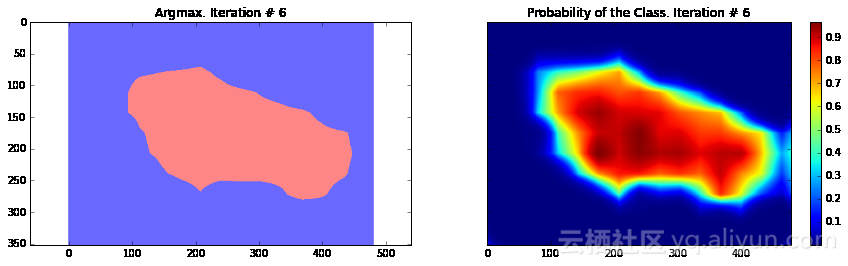Current Loss: 33199.1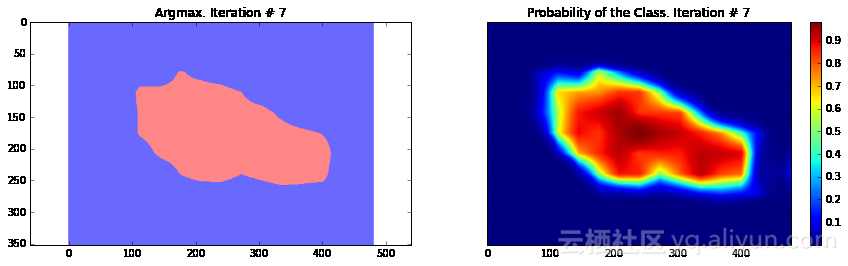Current Loss: 26540.3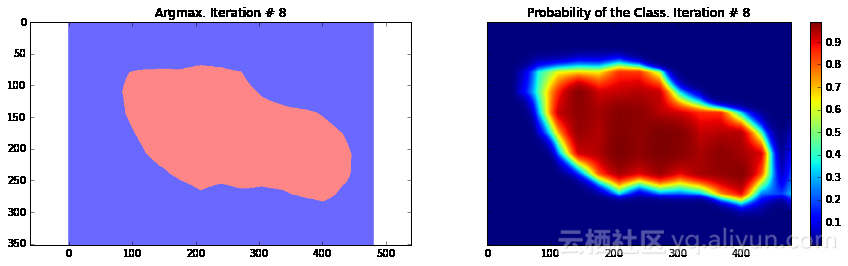Current Loss: 23658.0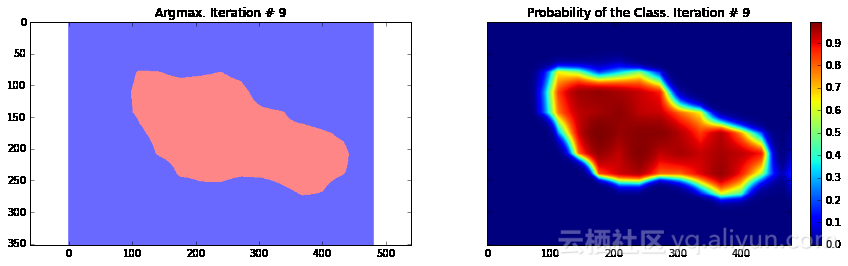Current Loss: 29404.9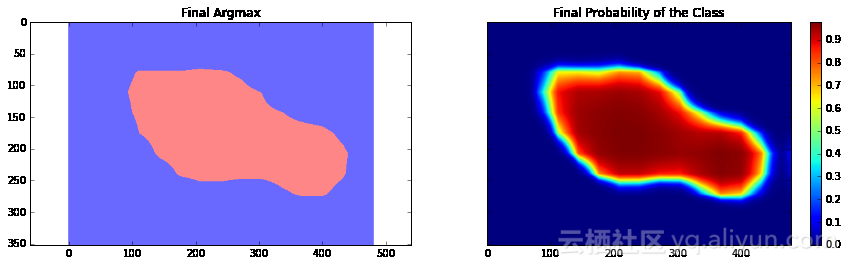Final Loss: 18177.5

正如你所看到的，结果非常粗糙——而且，这还是我们使用模型训练同一张图像来运行网络预测的结果。这在图像分割中一个非常常见的问题——分割结果通常很粗糙。有几种不同的方法可以解决此类问题——其中之一便是使用跳跃连接。主要思路是根据融合网络中不同层的预测结果来确定模型的最终预测结果。由于在网络较靠前的层次中下采样因子较小，所以，可以根据这些层来确定预测结果，进而取得更好的定位效果。Long等人发表的论文《Fully convolutional networks for semantic segmentation》介绍了这种方法。基于这种方法，研究人员设计了FCN-16s与FCN-8s架构。

另一种方法则基于带孔卷积与全连接条件随机场。Chen等人发表的论文《Semantic Image Segmentation with Deep Convolutional Nets and Fully Connected CRFs》介绍了这种方法。在本篇文章中，我们将仅使用条件随机场后处理阶段来展示它对模型效果的提高。

还有一点需要注意的是，当前模型训练时在全连接层（我们映射到卷积层的全连接层）中应用了Dropout技术，这种方法在Srivastava等人发表的论文《Dropout: a simple way to prevent neural networks from overfitting》中有所提及。Dropout是一种用于模型训练的正则化技术。它有一个非常优秀的理论描述，而且实现起来也非常简单:我们只需要在每个训练步骤中随机地选择一定数量的神经元，仅根据这些神经元来进行推断并后向传播。但是，从理论角度来看，Dropout可以看作是通过权重共享来训练一个稀疏网络的集合，每个网络仅进行很少次数的训练。在测试阶段，我们对所有这些网络的预测结果求均值。在论文中作者表明，Dropout在线性回归情况下预期能够取得与岭回归相同的效果。在我们的具体情况中，Dropout仅用于全连接层（我们映射到卷积层的全连接层）。这也解释了为什么最终模型的损失几乎比最后一次迭代的损失小了两倍——因为在最后的推断中，我们使用了损失的均值。

上面提供的代码用于处理单张图像，但你可以很容易地在整个数据集上运行这些程序。唯一需要调整的是，在每个迭代步骤中提供不同的图像。这种训练方式与论文《Fully convolutional networks for semantic segmentation》的做法完全一致，其中，论文作者采用了数量为1的批处理进行训练。

总的来说，我们可以看到我们的分割结果仍然很粗糙，需要执行一些额外的处理步骤。在下一节，我们将应用条件随机场的后处理步骤使模型的分割粒度更细。

条件随机场后处理

条件随机场是图模型的一种特定类型。在我们的应用场景中，条件随机场有助于根据网络的预测以及图像原生的RGB特征估计模型预测结果的后验分布。它通过最小化用户定义的能量函数来实现这一点。在我们的应用场景中，其效果与双边滤波器非常接近，双边滤波器综合考虑了图像中像素的空间邻近性以及在RGB特征空间（强度空间）中的相似性。

在一个非常简单的层面上，这种方法使用RGB特征来使图像分割结果更加精准——例如，边界通常表示为极大的强度变化——这是一个关键因素，位于该边界两侧的物体属于不同的类别。这种方法也同样对小的分割区域进行惩罚——例如，20像素或50像素大小的小区域通常不可能是一个正确的分割区域。物体通常由大的空间相邻的区域表示。

下面你可以看到这个后处理步骤是如何影响我们的分割结果的。我们使用了论文《fully connected crfs with gaussian edge potentials》有效性推断章节中描述的全连接条件随机场对分割结果进行调整。

在这部分中，我使用了一个版本稍微有些老的全连接条件随机场的实现，你可以在这里找到它。
import sys

path = "/home/dpakhom1/dense_crf_python/"
sys.path.append(path)

import pydensecrf.densecrf as dcrf

from pydensecrf.utils import compute_unary, create_pairwise_bilateral, \
create_pairwise_gaussian, softmax_to_unary

import skimage.io as io

image = train_image

softmax = final_probabilities.squeeze()

softmax = processed_probabilities.transpose((2, 0, 1))

# 输入数据应为概率值的负对数
# 你可以在softmax_to_unary函数的定义中找到更多信息
unary = softmax_to_unary(processed_probabilities)

# 输入数据应为C-连续的——我们使用了Cython封装器
unary = np.ascontiguousarray(unary)

d = dcrf.DenseCRF(image.shape * image.shape, 2)

d.setUnaryEnergy(unary)

# 潜在地对空间上相邻的小块分割区域进行惩罚——促使产生更多空间连续的分割区域
feats = create_pairwise_gaussian(sdims=(10, 10), shape=image.shape[:2])

d.addPairwiseEnergy(feats, compat=3,
kernel=dcrf.DIAG_KERNEL,
normalization=dcrf.NORMALIZE_SYMMETRIC)

# 这将创建与颜色相关的图像特征——因为我们从卷积神经网络中得到的分割结果非常粗糙，
# 我们可以使用局部的颜色特征来改善分割结果
feats = create_pairwise_bilateral(sdims=(50, 50), schan=(20, 20, 20),
img=image, chdim=2)

d.addPairwiseEnergy(feats, compat=10,
kernel=dcrf.DIAG_KERNEL,
normalization=dcrf.NORMALIZE_SYMMETRIC)
Q = d.inference(5)

res = np.argmax(Q, axis=0).reshape((image.shape, image.shape))

cmap = plt.get_cmap('bwr')

f, (ax1, ax2) = plt.subplots(1, 2, sharey=True)
ax1.imshow(res, vmax=1.5, vmin=-0.4, cmap=cmap)
ax1.set_title('Segmentation with CRF post-processing')
probability_graph = ax2.imshow(np.dstack((train_annotation,)*3)*100)
ax2.set_title('Ground-Truth Annotation')
plt.show()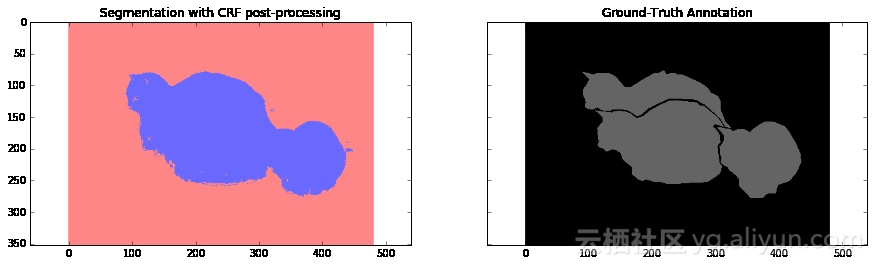结论与讨论

在本篇教程中，我们看到了卷积神经网络应用于图像分割领域时的一个缺陷——粗糙的分割结果。我们可以看到，这种情况是由于在VGG-16网络架构中使用最大值池化层所导致的。

根据像素间交叉熵损失的定义，我们在简化的场景下进行了模型的训练，并使用后向传播来更新权重。

我们使用条件随机场（CRFs）来解决分割结果粗糙的问题，并取得了很好的效果。

以下为译文

**本文由北邮@爱可可-爱生活 老师推荐，阿里云云栖社区组织翻译。

原标题《Image Segmentation with Tensorflow using CNNs and Conditional Random Fields》，作者：Daniil，译者：6816816151**

文章为简译，更为详细的内容，请查看原文


展开全文• 在模型参数上,提出了三种不同学习率和三种优化函数进行精确的实验对比,绘制了不同学习率和优化函数的参数对比,根据实验结果找到最优的卷积神经网络模型学习率和优化函数参数。对实验结果的分析表明,该方法在受试者...
• 针对深度卷积神经网络中 maxout 单元非最大特征无法传递、特征图像子空间池化表达能力不足局限性，提出混合maxout (mixout,mixed maxout)单元。首先，计算相同输入在不同卷积变换所形成特征图像子空间指数...深度学习 maxout单元 激活函数
• 基于卷积神经网络的腹腔镜软组织器官表面无监督学习深度估计网络，并根据视差图像进行三维重建。 实验结果表明，提出深度估计网络所计算产生视差图像为稠密有效根据视差图像进行三维重建结果较好
•   终于进入我们主题了ConvNets或者CNNs，它结构和普通神经网络都一样，之前我们...首先我们分析传统神经网络对于图片处理，如果还是用CIFAR-10上图片，共3072个特征，如果普通网络结构输入那么第一层...


终于进入我们的主题了ConvNets或者CNNs，它的结构和普通神经网络都一样，之前我们学习的各种技巧方法都适用，其主要不同之处在于：
ConvNet假定输入的是图片，我们根据图片的特性对网络进行设定以达到提高效率，减少计算参数量的目的。

1. 结构总览

首先我们分析下传统神经网络对于图片的处理，如果还是用CIFAR-10上的图片，共3072个特征，如果普通网络结构输入那么第一层的每一个神经单元都会有3072个权重，如果更大的像素的图片进入后参数更多，而且用于图片处理的网络一般深度达10层之上，加在一起参数的量实在太大，参数过多也会造成过拟合，而且图片也有自身的特点，我们需要利用这些特点，将传统网络改革，加快处理速度和精确度。
我们注意到图片的像素是由3个通道构成的，我们就利用了这个特点将其神经元安置到了三维空间（width, height, depth），分别对应着图片的32x32x3（以CIFAR为例）如下图：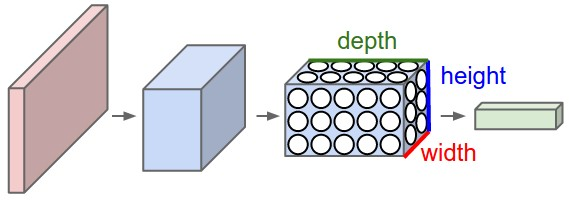红色是输入层这里的深度是3，输出层是1x1x10的结构。其他几层的含义后面会介绍，现在先知道每层都是height × width × depth结构。

2. 卷积神经网络的层

卷积神经网络有三种层：卷积层、池化层和全连接层（Convolutional Layer, Pooling Layer, 及 Fully-Connected Layer）。
以处理CIFAR-10的卷积神经网络为例，简单的网络应包含这几层：
[INPUT - CONV - RELU - POOL - FC]也就是[输入-卷积-激活-池化-分类得分]，各层分述如下：

INPUT [32x32x3] 输入长32宽32带有三个通道的图片
CONV ：计算图片的局部区域，如果我们想要使用12个过滤器fliters，他的体积将是 [32x32x12].
RELU ：还是一个激励层max(0,x) ，尺寸还是 ([32x32x12]).
POOL： 沿着图片的(width, height)采样, 减小长宽的维度，例如得到的结果是 [16x16x12].
FC (i.e. fully-connected) 计算分类得分最终尺寸是 [1x1x10], 这层是全连接的，每一个单元都与前一层的各个单元连接。
注意：
1. 卷及神经网络包含不同的层 (e.g. CONV/FC/RELU/POOL 也是最受欢迎的)
2. 每一层都输入输出3d结构的数据，除了最后一层
3. 有些层可能没有参数，有些层可能有参数 (e.g. CONV/FC do, RELU/POOL don’t)
4. 有些层可能有超参数有些层也可能没有超参数(e.g. CONV/FC/POOL do, RELU doesn’t)
下图是一个例子，没法用三维表示只能展成一列的形式了。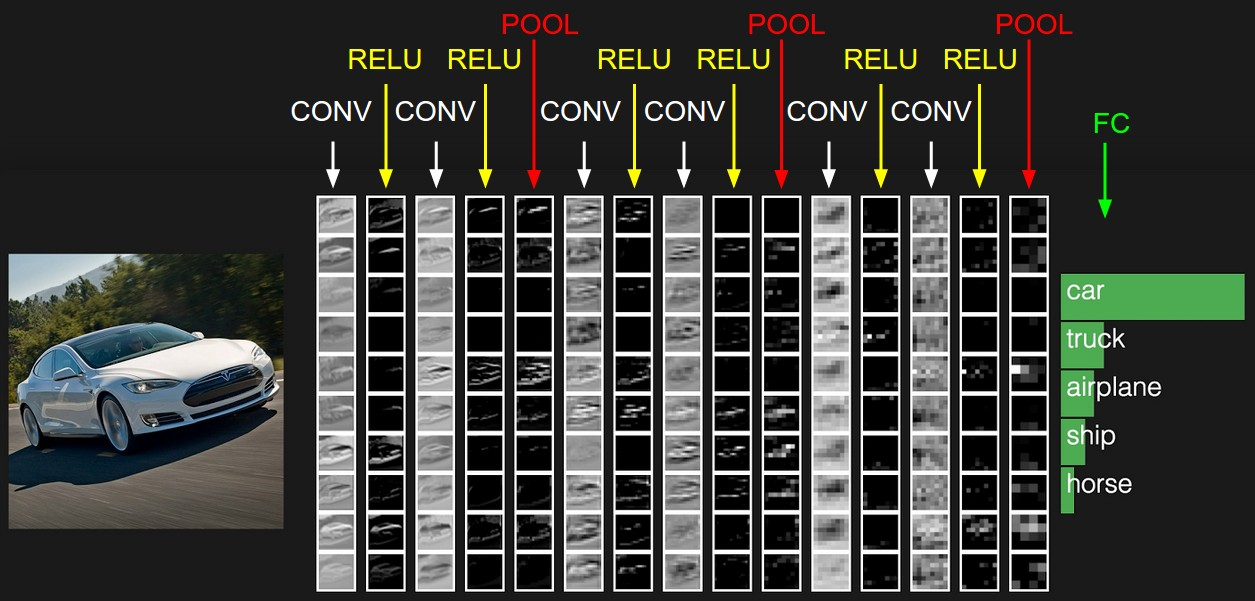下面展开讨论各层具体细节：

2.1 卷积层

卷积层是卷积神经网络的核心层，大大提高了计算效率。
卷积层由很多过滤器组成，每个过滤器都只有一小部分，每次只与原图像上的一小部分连接，UFLDL上的图：这是一个过滤器不停滑动的结果，
我们这里要更深入些，我们输入的图像是一个三维的，那么每个过滤器也是有三个维度，假设我们的过滤器是5x5x3的那么我们也会得到一个类似于上图的激活值的映射也就是convolved feature 下图中叫作 activion map,其计算方法是wT×x+b

其中w是5x5x3=75个数据，也就是权重，他是可以调节的。
我们可以有多个过滤器：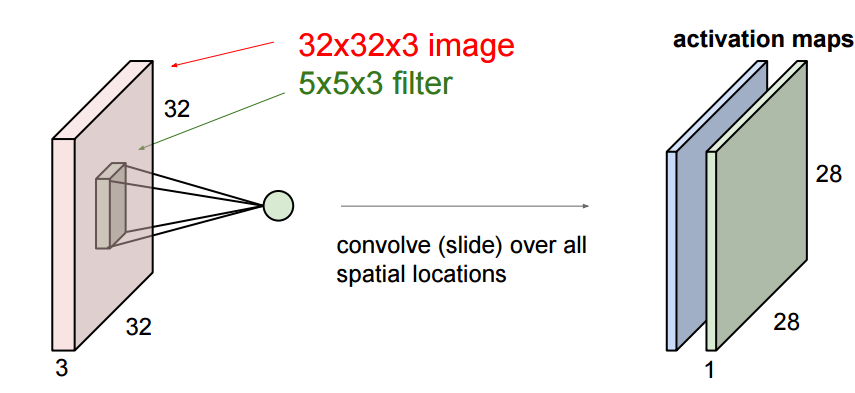更深入一些，当我们滑动的时候有三个超参数：
1. 深度，depth，这是过滤器的数量决定的。
2. 步长，stride，每次滑动的间隔，上面的动画每次只滑动1个数，也就是步长为1.
3. 补零数， zero-padding，有时候根据需要，会用零来拓展图像的面积，如果补零数为1，变长就＋2，如下图中灰色的部分就是补的0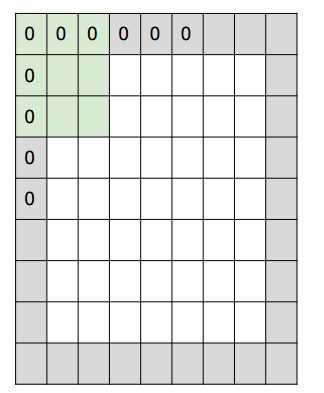下面是一个一维的例子：其输出的空间维度计算公式是

(W−F+2P)/S+1

其中w是输入的尺寸，f是过滤器的尺寸，p是补零的尺寸，s是步长，图中如果补零为1那么输出为5个数，步长为2输出为3个数。
到现在为止我们好像都没有涉及到神经这个概念哇，现在我们就从神经角度来理解：
上面提到每一个激活值都是：wT×x+b，这个公式我们熟悉哇，这就是神经元的得分公式呀，所以我们可以将每一个 activation map 其看做是一个filter的杰作，如果有5个过滤器，就会有5个不同的filter同时连接一个部分。
卷积神经网络还有一个重要的特征：权重共享：在同一个filter上的不同的神经单元（滑动窗口）的权重是相同的。这样以来大大减少了权重的数量。
这样每一层权重相同，每一个过滤器计算的结果都是一个卷积（后来还会加一个偏差b）: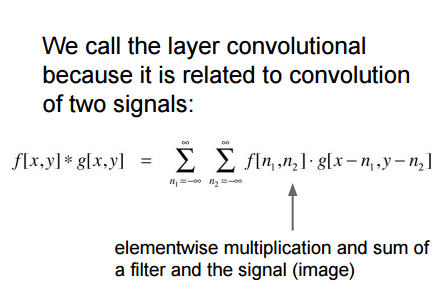这也是卷积神经网络的名字的来源。
下面这张图是错误的，请看官网http://cs231n.github.io/convolutional-networks/#conv，找出其中的错误就明白卷积的工作原理了。（gif来自寒小阳的博客）
虽然这里吧每一个filter的权重w变为而来三部分，但是在神经元里还是用了wx+b的形式。
- 反向传播：这种卷积的反向传播还是卷积，计算过程也比较简便
- 1x1 convolution：一些文章用了1*1的卷积, 比如最开始的Network in Network. 这样可以有效的做多个内积， 输入有三层，那么每层至少要有三个w，也就是把上面的动态图的filter变为1x1x3.
-Dilated convolutions.最近又有研究(e.g. see paper by Fisher Yu and Vladlen Koltun) 对卷积层添加了一个超参数：dilation。这是对filter的进一步控制，我们开一下效果：dilation等于0时，这样计算卷积wx + wx + wx；dilation =1时变成了这样wx + wx + wx;也就是我们要处理的图像中每个隔了个1. 这就允许了利用更少的层来融合空间信息. 例如我们用了两个 3x3 CONV layers在最顶层 ，这是第二层起到了 感受5x5 （ effective receptive field）的作用. 如果使用dilated convolutions 那么这个effective receptive field会以指数形式增长.

2.2 池化层

上面可以知道在卷积层之后得到的结果还是挺多，而且由于滑动窗口的存在，很多信息也有重合，于是有了池化pooling 层，他是将卷积层得到的结果无重合的分来几部分，然后选择每一部分的最大值，或者平均值，或者2范数，或者其他你喜欢的值，我们以取最大值的max pool为例：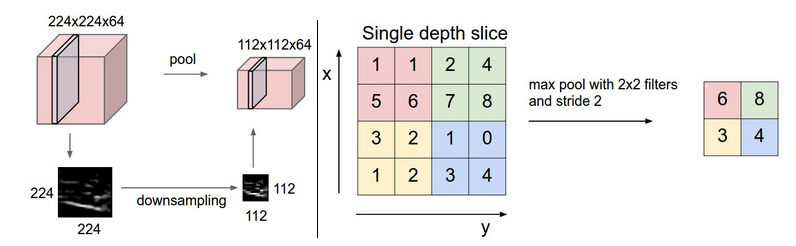- 反向传播：最大值的梯度我们之前在反向传播的时候就已经学习过了，这里一般都跟踪最大的激活值，这样在反向传播的时候会提高效率.
- Getting rid of pooling. 一些人认为pooling是没有必要的，如The All Convolutional Net ，很多人认为没有池层对于生成式模型（generative models）很重要，似乎以后的发展中，池层可能会逐渐减少或者消失。

2.3 其他层

Normalization Layer，以前的时候用normalization 层的模拟人脑的抑制作用，但是逐渐认为没有多大的帮助，于是用的少了，这篇论文里有介绍其作用 Alex Krizhevsky’s cuda-convnet library API.
Fully-connected layer，这个全连接层和以前学过的一样，前面提到过最后的分类层是全连接层。
2.4 Converting FC layers to CONV layers

全连接层和卷积层除了连接方式不一样，其计算方式都是内积，可以相互转换：
1. FC如果做CONV layer 的工作就相当于其矩阵的多数位置都是0（稀疏矩阵）。
2. FC layer 如果被转变为 CONV layer. 相当于每一层的局部连接变为了全部链接如FC layer with K=4096的输入是7×7×512那么对应的卷积层为 F=7,P=0,S=1,K=4096输出为1×1×4096。
例子：
假设一个cnn输入 224x224x3图像,经过若干变化之后某一层输出 7x7x512 到这里之后使用两4096的FC layer及最后一个1000的FC计算分类得分下面是把这三层fc转化为Conv 的过程:
1. 使用 F=7的conv layer 输出为 [1x1x4096]；
2. 使用F=1的过滤器，输出为 [1x1x4096]；
3. 使用F=1的卷积层，输出为 [1x1x1000]。

每次转化都会将FC的参数转变为conv的参数形式. 如果在转变后的系统中传入更大的图片，也会非常快速的向前运算。例如将384x384的图像输入上面的系统，会在最后三层之前得到[12x12x512]的输出, 经过上面转化的conv 层会得到 [6x6x1000], （(12 - 7)/1 + 1 = 6）. 我们一下就得出了6x6的分类结果。
这样一次得到的比原来使用迭代36次得到的要快。这是实际应用中的技巧。
另外我们可以用两次步长16的卷积层代替一次步长为32的卷积层来输入上面的图片，提高效率。

3 搭建卷积神经网络

下面我们就用CONV, POOL，FC ，RELU 搭建一个卷积神经网络：

3.1 层次结构

我们按照以下结构搭建

INPUT -> [[CONV -> RELU]*N -> POOL?]*M -> [FC -> RELU]*K -> FC

1
其中N >= 0 (一般N <= 3), M >= 0, K >= 0 (一般K < 3).这里要注意：我们更倾向于使用多层小size的CONV。
为什么呢？
比如3个3x3的和一个7x7的conv层，他们都可以 得到7x7的receptive fields.但是3x3的有以下优点：
1. 3层的非线性组合要比一层线性组合表达能力强；
2. 3层小尺寸的卷积层的参数数量要少，3x3x3<7x7；
3. 反向传播中我们需要使用更多的内存来储存中间层结果。

值得注意的是Google’s Inception architectures 及Residual Networks from Microsoft Research Asia. Both 等创造了相比以上结构更加复杂的连接结构。

3.2 层的大小

输入层：输入层一般是2的指数形式比如 32 (e.g. CIFAR-10), 64, 96 (e.g. STL-10), or 224 (e.g. common ImageNet ConvNets), 384, 512等.
卷积层：一般是小的过滤器比如3x3或者最大 5x5，步长设为1，当加入补零时卷积层可能不会改变输入的尺寸，如果必须使用大的过滤器，那么经常在第一层使用补零的方法P=(F−1)/2。
池化层：常见的设置是使用2x2的最大池化层，很少有超过3x3的最大池化层。
如果我们的步长大于1或者没有补零，我们需要非常注意看是否我们的步长和顾虑器是否足够健壮，我们的网络是否均匀对称连接。
步长为1表现更好，对池化也更有兼容新.
补零的好处：如果不补零那么边缘的信息会很快被抛弃
要考虑到电脑的内存限制。例如输入 224x224x3 图片，过滤器为 3x3 共64 filters，padding 为1 这样每张图片需要72MB 内存 ，但是GPU上运行的话，内存可能不够所以可能会调整下参数比如 filter为 7x7，stride 为 2 (ZF net). 或者filer11x11 ， stride of 4.（AlexNet）
3.3 案例

LeNet. 第一个成功应用的cnn（ Yann LeCun in 1990’s）. 他的强项是杜zip codes, digits, etc.
AlexNet. 第一个广泛的应用于计算机视觉, （by Alex Krizhevsky, Ilya Sutskever and Geoff Hinton）. ImageNet ILSVRC challenge in 2012 大放异彩，与LeNet结构类似不过更深更大，多层卷积层叠加。
ZF Net. The ILSVRC 2013 winner （ Matthew Zeiler and Rob Fergus）. It became known as the ZFNet (short for Zeiler & Fergus Net).调整了Alexnet的结构参数, 扩大了中间卷积层 使第一层的过滤器和步长都减小了.
GoogLeNet. The ILSVRC 2014 winner（Szegedy et al. from Google.） 极大的减少了参数数量 (由 60M到4M). 使用Average Pooling代替了 ConvNet的第一个FC层, 消除了大量的参数，有很多的变体如：Inception-v4.
VGGNet. The runner-up in ILSVRC 2014 （ Karen Simonyan and Andrew Zisserman ）证明了深度的好处. 可以在Caffe上使用. 但是参数太多，(140M)，计算量大 . 不过现在已经正实有很多不需要的参数可以去除。
ResNet. （Kaiming He et al）.winner of ILSVRC 2015. 截止2016年5月10，这是一种最先进的模型. 现在也有了改进版 Identity Mappings in Deep Residual Networks (published March 2016).
其中VGG的计算花费为：
INPUT: [224x224x3]        memory:  224*224*3=150K   weights: 0
CONV3-64: [224x224x64]  memory:  224*224*64=3.2M   weights: (3*3*3)*64 = 1,728
CONV3-64: [224x224x64]  memory:  224*224*64=3.2M   weights: (3*3*64)*64 = 36,864
POOL2: [112x112x64]  memory:  112*112*64=800K   weights: 0
CONV3-128: [112x112x128]  memory:  112*112*128=1.6M   weights: (3*3*64)*128 = 73,728
CONV3-128: [112x112x128]  memory:  112*112*128=1.6M   weights: (3*3*128)*128 = 147,456
POOL2: [56x56x128]  memory:  56*56*128=400K   weights: 0
CONV3-256: [56x56x256]  memory:  56*56*256=800K   weights: (3*3*128)*256 = 294,912
CONV3-256: [56x56x256]  memory:  56*56*256=800K   weights: (3*3*256)*256 = 589,824
CONV3-256: [56x56x256]  memory:  56*56*256=800K   weights: (3*3*256)*256 = 589,824
POOL2: [28x28x256]  memory:  28*28*256=200K   weights: 0
CONV3-512: [28x28x512]  memory:  28*28*512=400K   weights: (3*3*256)*512 = 1,179,648
CONV3-512: [28x28x512]  memory:  28*28*512=400K   weights: (3*3*512)*512 = 2,359,296
CONV3-512: [28x28x512]  memory:  28*28*512=400K   weights: (3*3*512)*512 = 2,359,296
POOL2: [14x14x512]  memory:  14*14*512=100K   weights: 0
CONV3-512: [14x14x512]  memory:  14*14*512=100K   weights: (3*3*512)*512 = 2,359,296
CONV3-512: [14x14x512]  memory:  14*14*512=100K   weights: (3*3*512)*512 = 2,359,296
CONV3-512: [14x14x512]  memory:  14*14*512=100K   weights: (3*3*512)*512 = 2,359,296
POOL2: [7x7x512]  memory:  7*7*512=25K  weights: 0
FC: [1x1x4096]  memory:  4096  weights: 7*7*512*4096 = 102,760,448
FC: [1x1x4096]  memory:  4096  weights: 4096*4096 = 16,777,216
FC: [1x1x1000]  memory:  1000 weights: 4096*1000 = 4,096,000

TOTAL memory: 24M * 4 bytes ~= 93MB / image (only forward! ~*2 for bwd)
TOTAL params: 138M parameters

1
2
3
4
5
6
7
8
9
10
11
12
13
14
15
16
17
18
19
20
21
22
23
24
25
26
27
注意到内存使用最多的时候在开始几个CONV layers, 参数基本都在最后几个FC层第一个FC有100M个！

3.4 内存使用

内存主要在以下几个方面消耗较多
1. 大量的激活值和梯度值。测试时可以只储存当前的激活值丢弃之前的在下方几层激活值会大大减少激活值的储存量。
2. 参数的储存，在反向传播时的梯度及使用 momentum, Adagrad, or RMSProp时的缓存都会占用储存，所以估计参数使用的内存时一般至少要乘以3倍
3. 每次网络运行都要记住各种信息比如图形数据的批量等
如果估计网络需要的内存太大，可以适当减小图片的batch，毕竟激活值占用了大量的内存空间。

其他资料

Soumith benchmarks for CONV performance
ConvNetJS CIFAR-10 demo 浏览器的ConvNets实时演示.
Caffe,流行的ConvNets工具
State of the art ResNets in Torch7

展开全文• 卷积神经网络基础知识（一）文前白话卷积神经网络的发展卷积神经网络的结构1、全连接层① 神经元概念② 隐层2、卷积层①卷积操作的过程②卷积操作的特点与注意点③ 附加条件下的卷积操作2、池化层① 池化操作方式② ...
卷积神经网络基础知识（一）文前白话卷积神经网络的发展卷积神经网络的结构1、全连接层① 神经元概念② 隐层2、卷积层①卷积操作的过程②卷积操作的特点与注意点③ 附加条件下的卷积操作3、池化层① 池化操作方式② 池化的特点
文前白话
这个系列根据哔站博主<神秘的wz>主讲的课程制作而成，主要以笔记的形式呈现，添加自己的理解以及一些思维导图，巩固所学。文末附有视频链接。
卷积神经网络的发展

卷积神经网络的发展简史：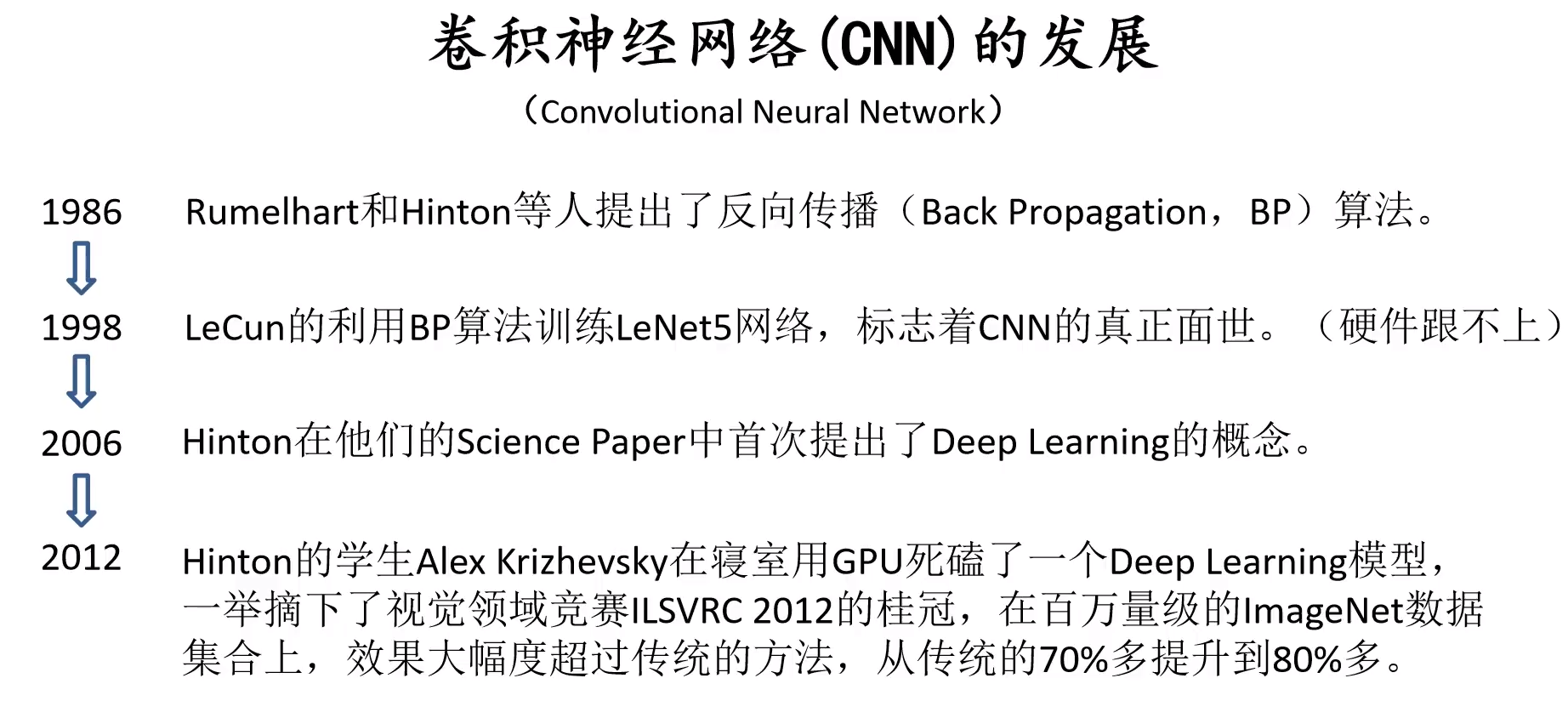反向传播机制：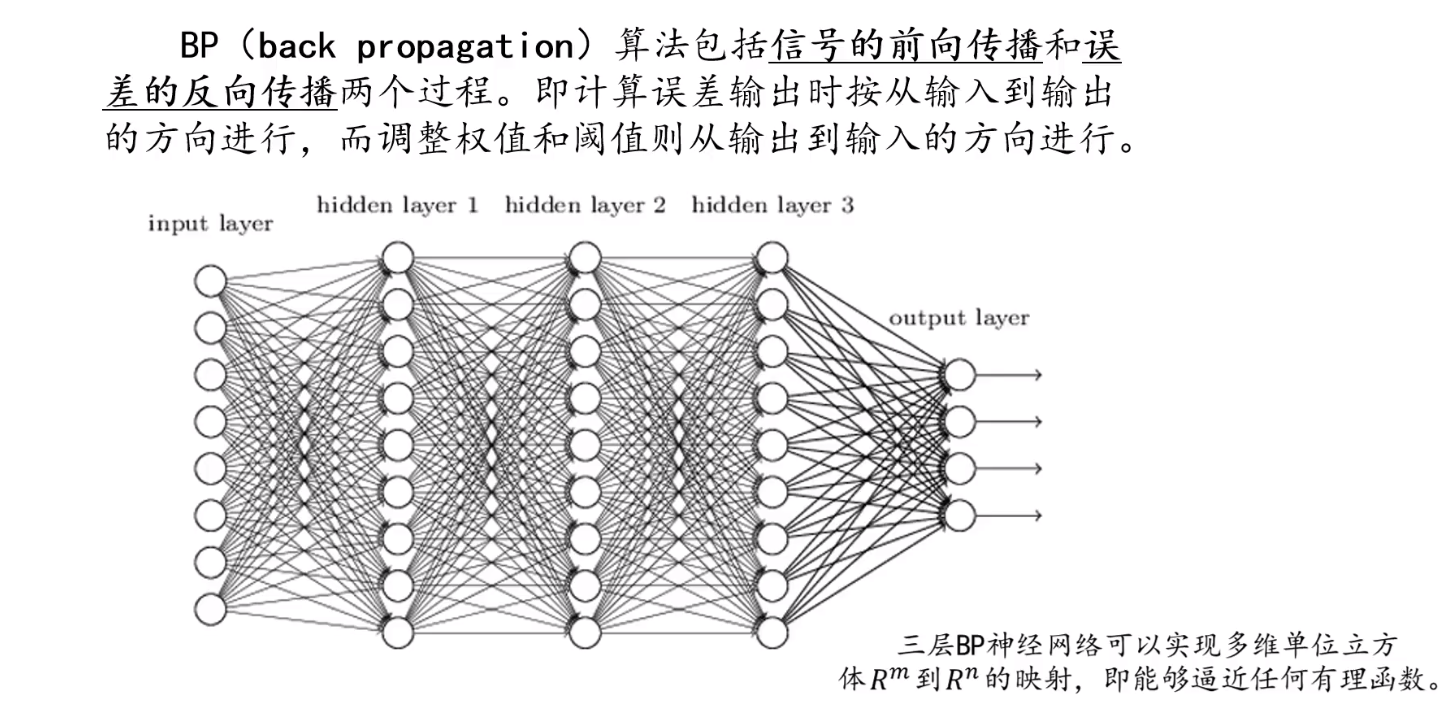LeNet 网络结构：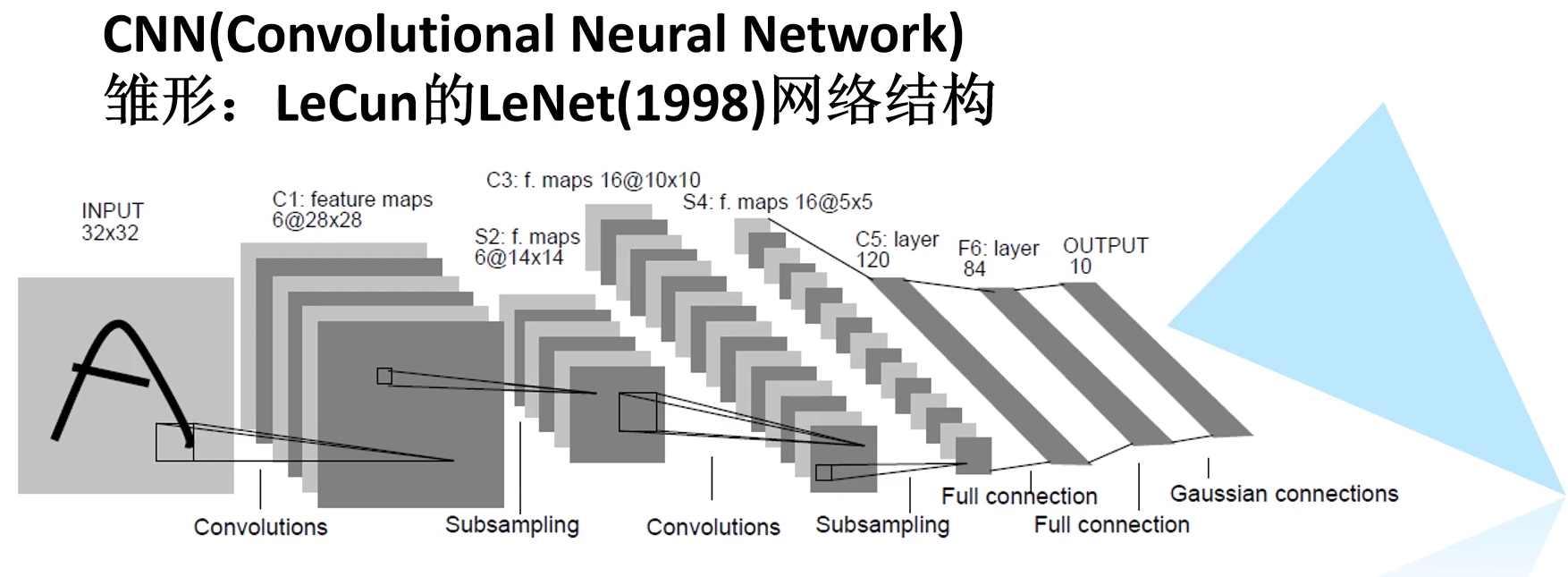卷积神经网络广泛应用于图像检测、分类、分割等等方面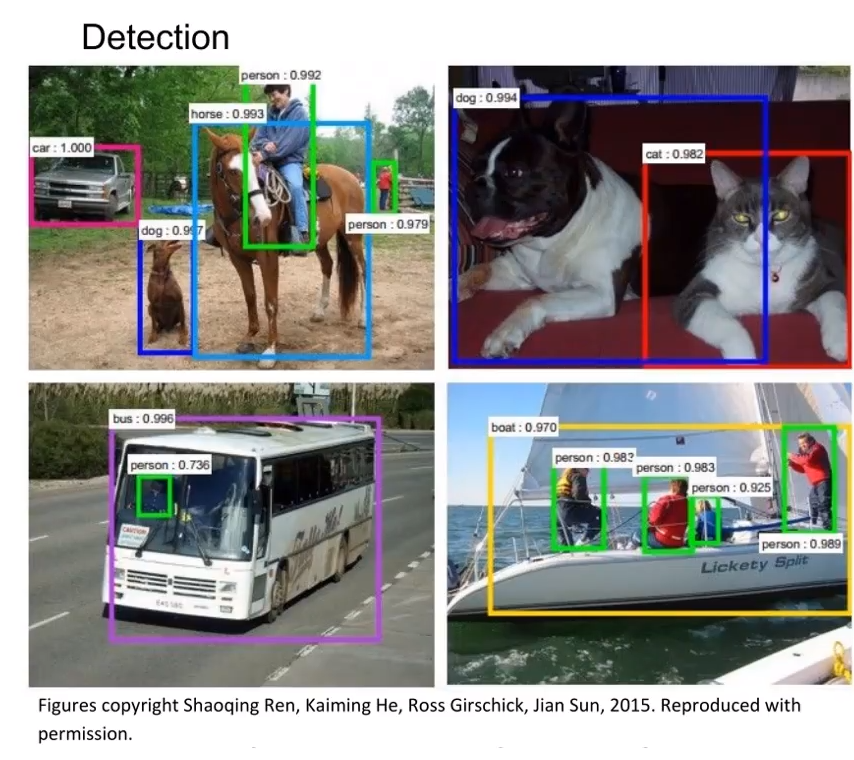卷积神经网络的结构
1、全连接层
① 神经元概念

基本概念： 神经元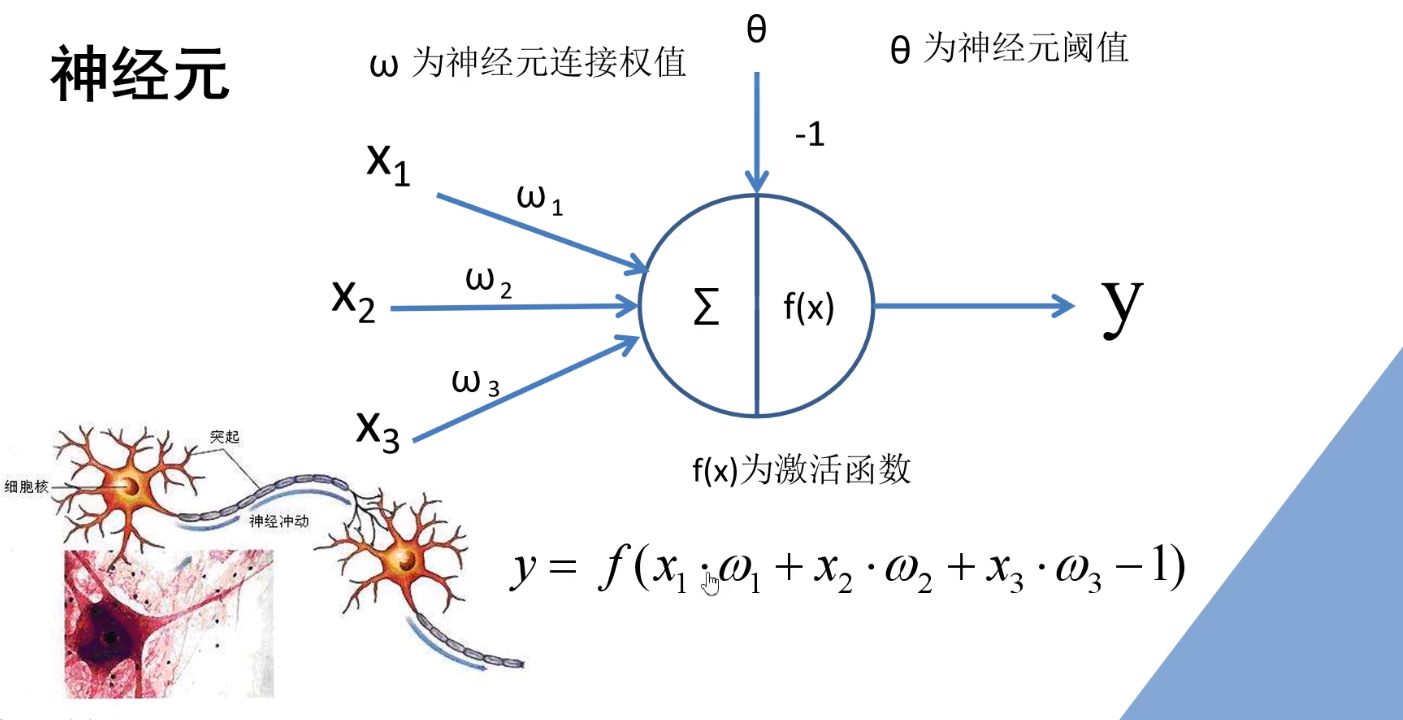② 隐层

神经网络中，输入层神经元只是接受输入，不进行函数处理，所以输入层不计入层数，如果只有一个隐层，则称为单隐层神经网络。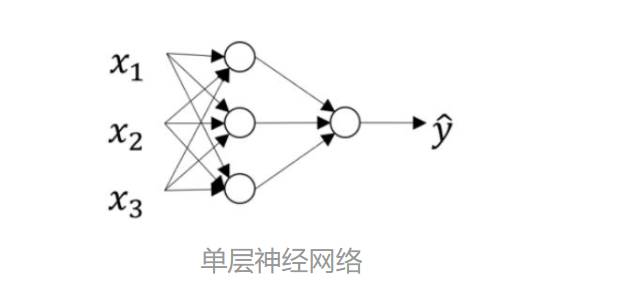如果只有一个隐层（两层神经元的神经网络），则称为单隐层神经网络。
如果两个隐层则称为双隐层神经网络。而只需包含隐层，就可称为多层神经网络。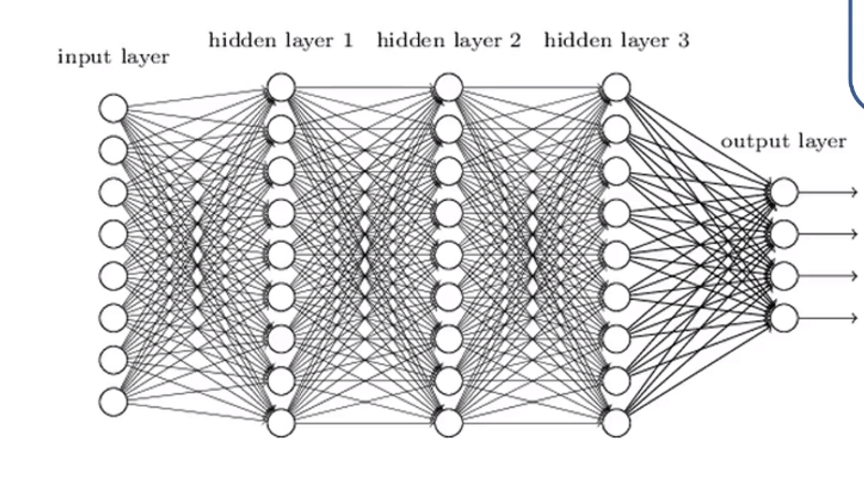用一个例子来理解  输入 / 输出 网络中的信息  的概念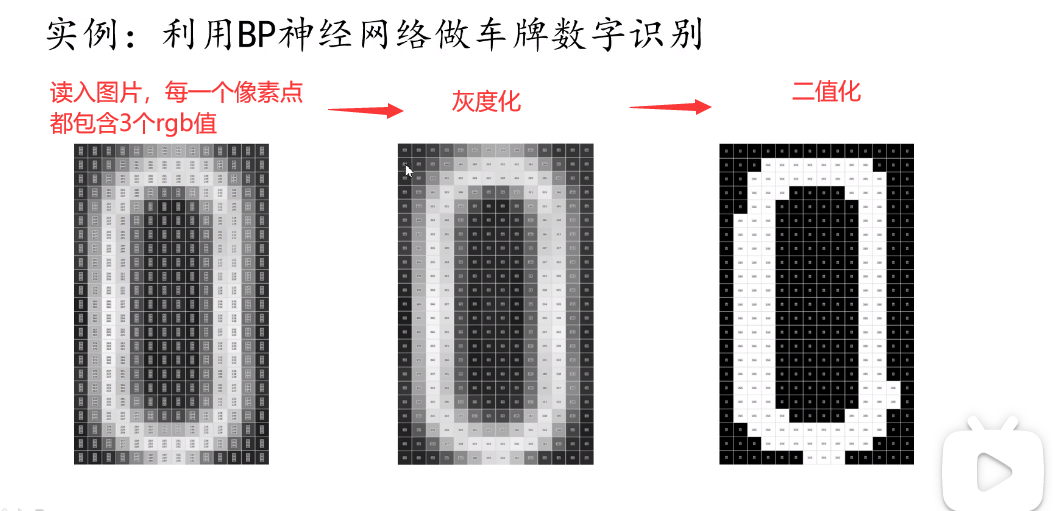① 输入图像，进行灰度化、二值化处理

注释： 原始图片有3个channel，灰度化处理以后图象只有一个channel了， 再进行二值化处理，可以理解为：二值化将像素点分为特征点和分特征点进行区分识别

② 进行特征提取：这里用用 5×3 的框进行滑移，计算白色像素点（特征点）占整个框像素点的比例， 对于边缘不够的填充框的部分进行补0处理（padding），或者提前进行预判，在边缘处重新设置框的大小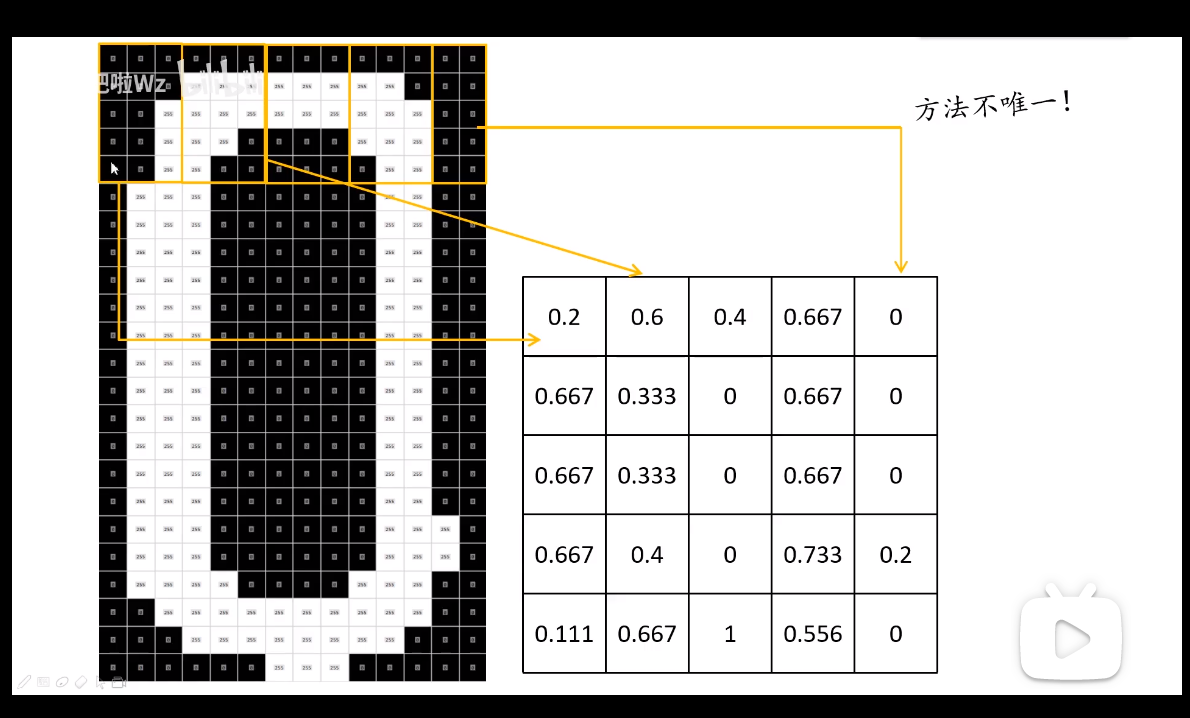③  对于得到的特征矩阵按行展开，得到一个 1×25 的矩阵，作为神经网络的输入
这里为什么要用行展开，可以进行深入的了解。

④ 神经网络的输出

先理解：
one-hot   编码方式：按照序列，需要表示几，相应的位置就是 1 ，其他位置都是 0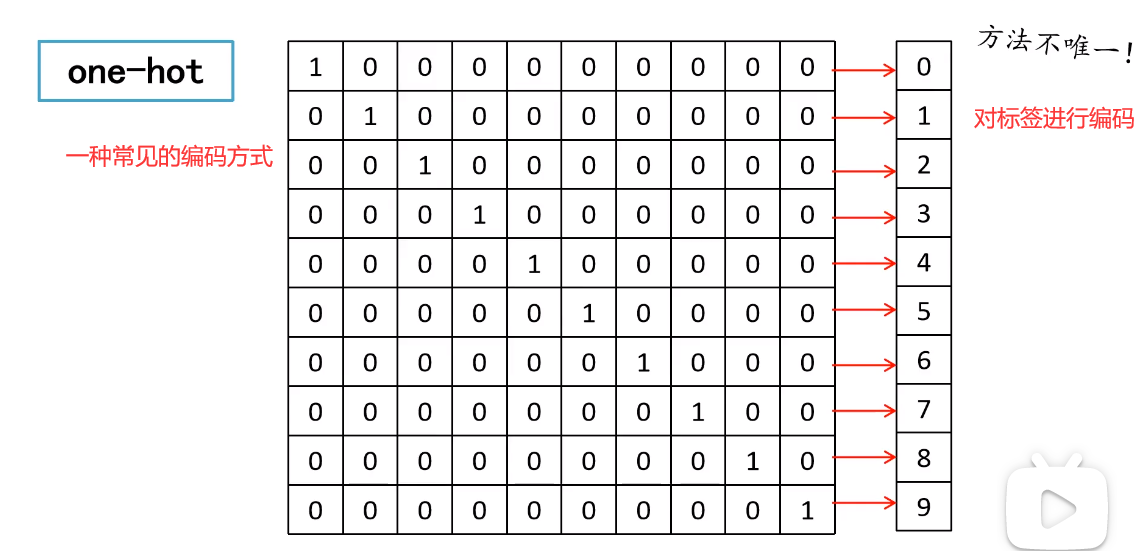这种编码方式，可以表示神经网络输出端的结果，有了输入的特征和期望的输出，就可以使用神经网络的框架进行训练，然后输出结果。
至于输入/输出的节点数可以根据实际的需求进行设定。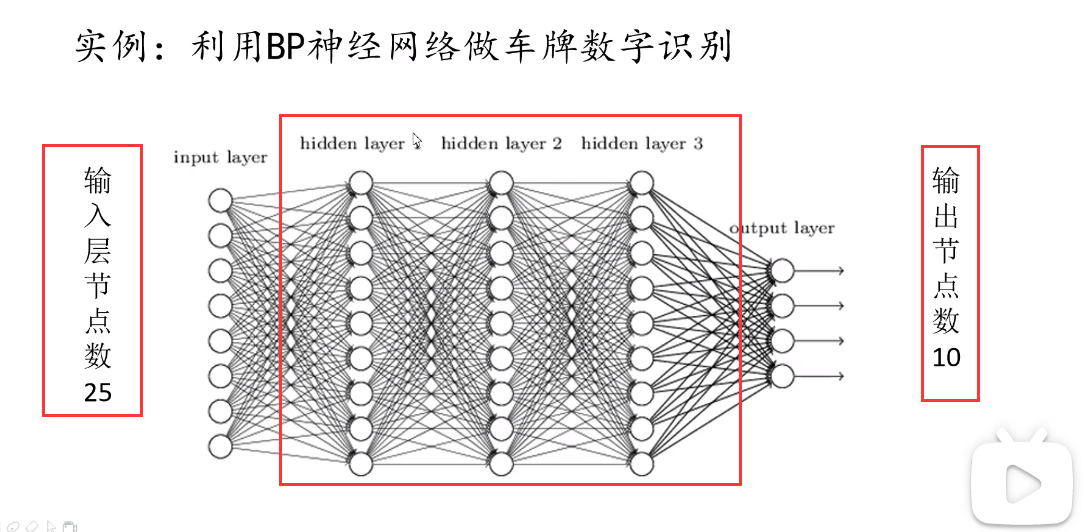2、卷积层
①卷积操作的过程

卷积的目的：对图像的特征进行提取
（上述的车牌识别案例中，用框平移，计算框内白色像素的比例就是卷积的实质过程）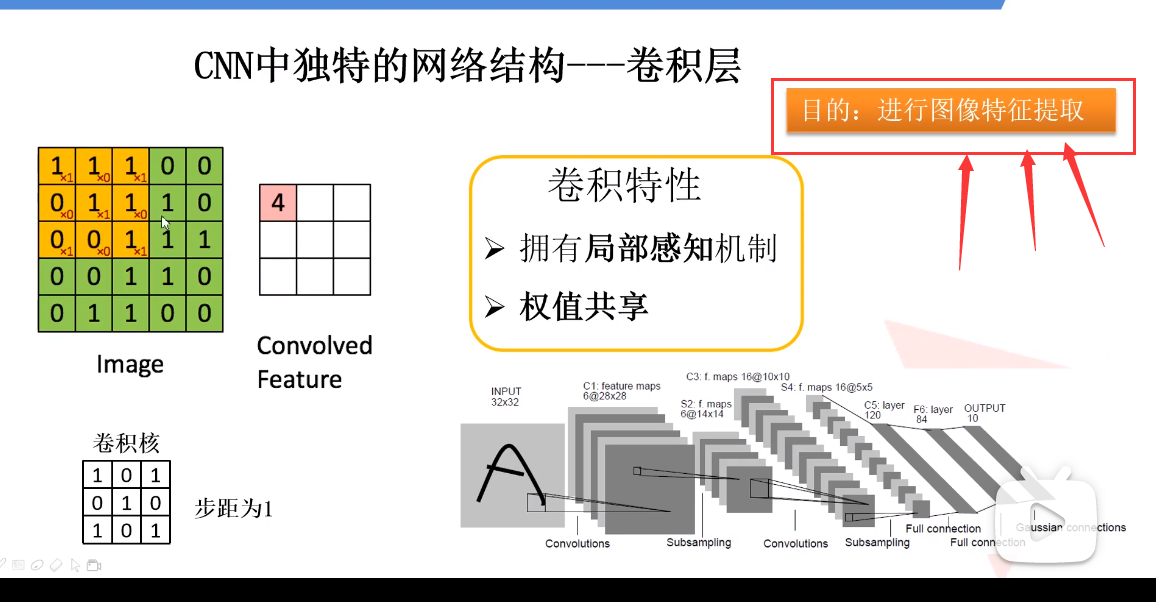进行卷积操作，需要提前选定好卷积核（滤波器）的大小， 就是框的大小，而且卷积操作的方式不唯一！！！
计算过程如下：对应位置相乘，再累加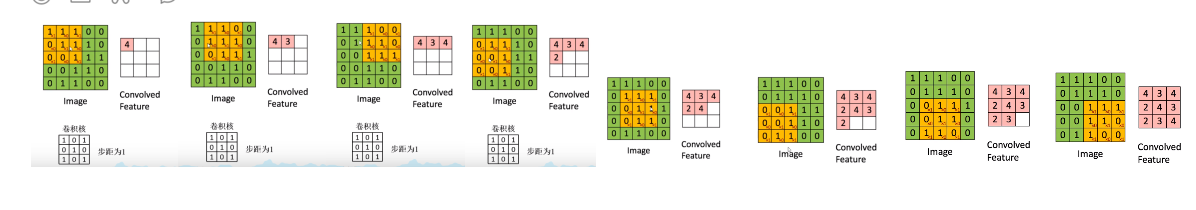②卷积操作的特点与注意点

卷积的特点：
①局部感知机
②权值共享（简化计算）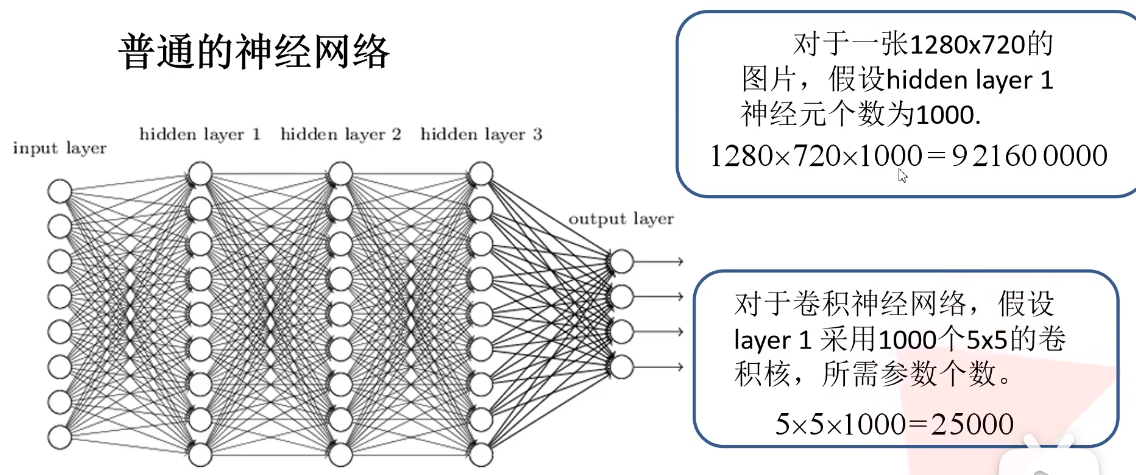卷积的注意点：

① 卷积核的channel与输入特征层的channel相同
② 输出的特征矩阵channel与卷积核的数目相同

实例：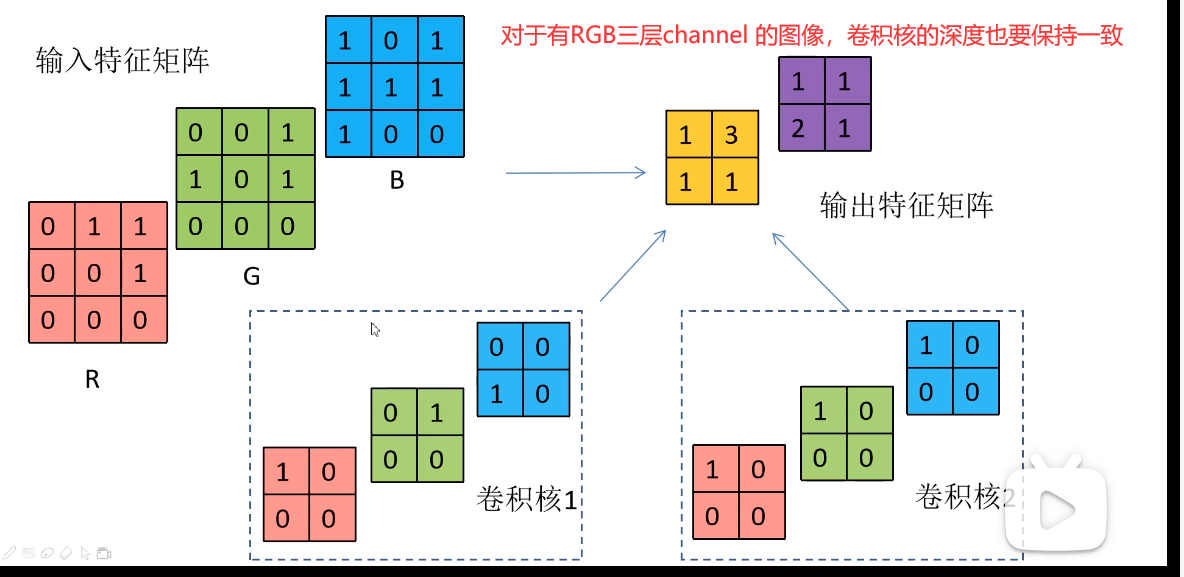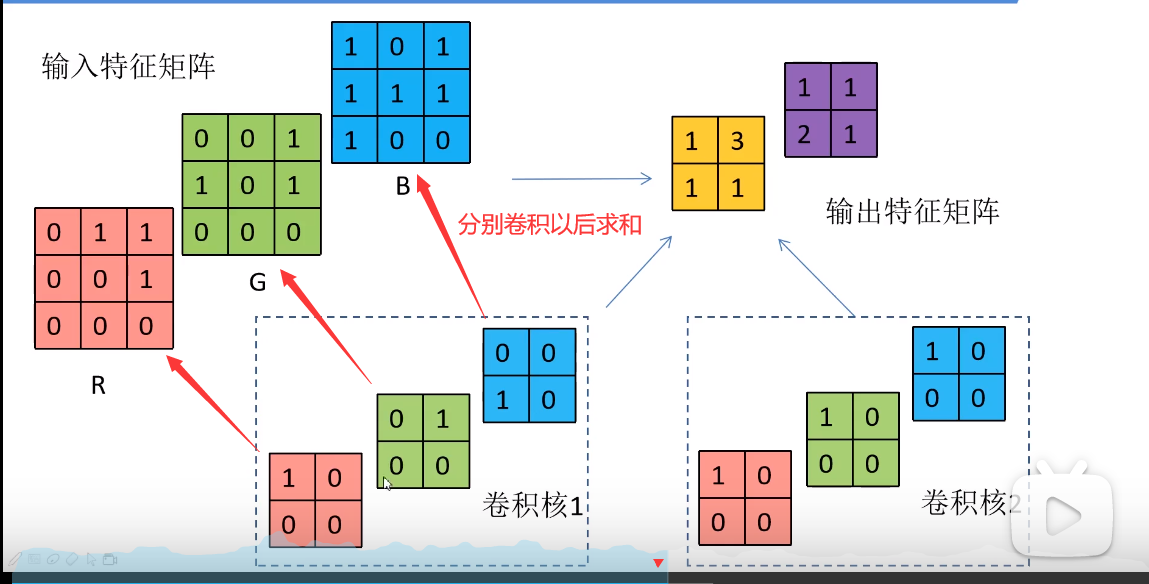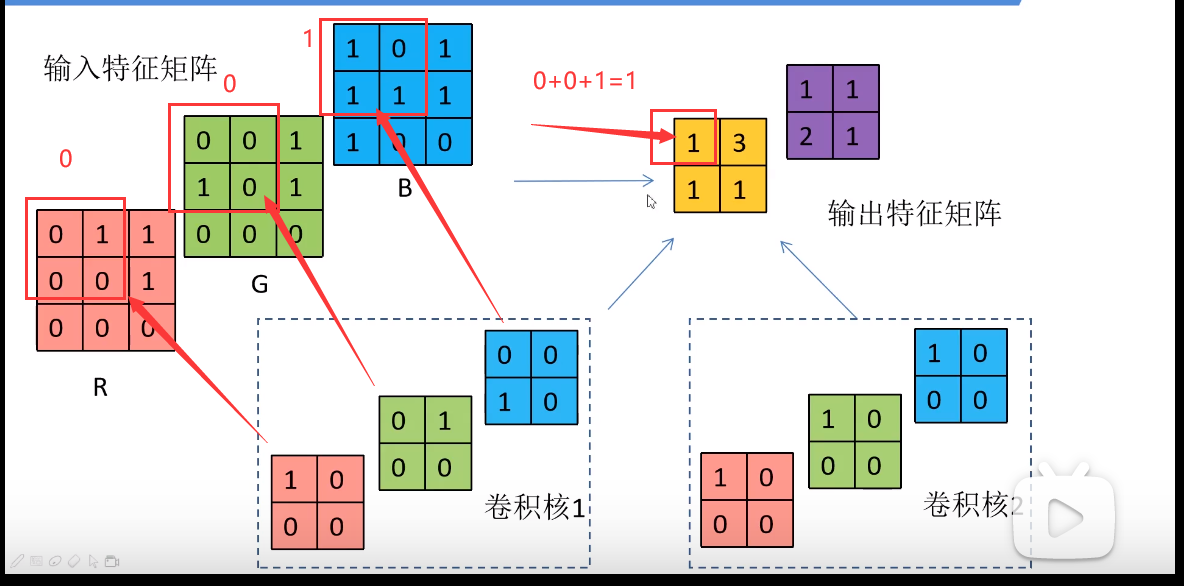③ 附加条件下的卷积操作

问题：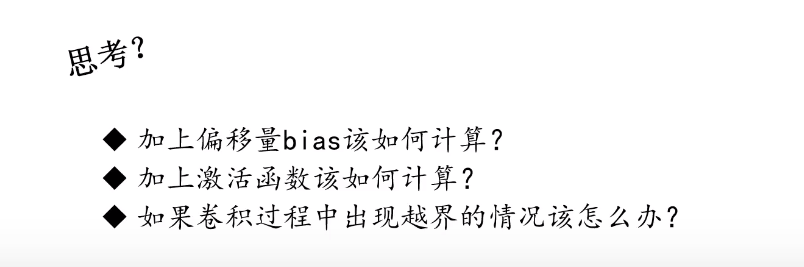解决方法：

① 对于偏移量的附件，直接在卷积得到的结果上进行运算相加减即可。

为什么要使用激活函数：
因为，线性组合的线性组合，还是线性组合。如果是线性激活函数，那么神经网络无论多少层，最后还是线性组合，而线性组合能解决的问题范围是很狭窄的。（深度学习的所谓深度就无法发挥作用了），所以，通过激活函数引入非线性因素，可以用来解决非线性问题。

常见的激活函数：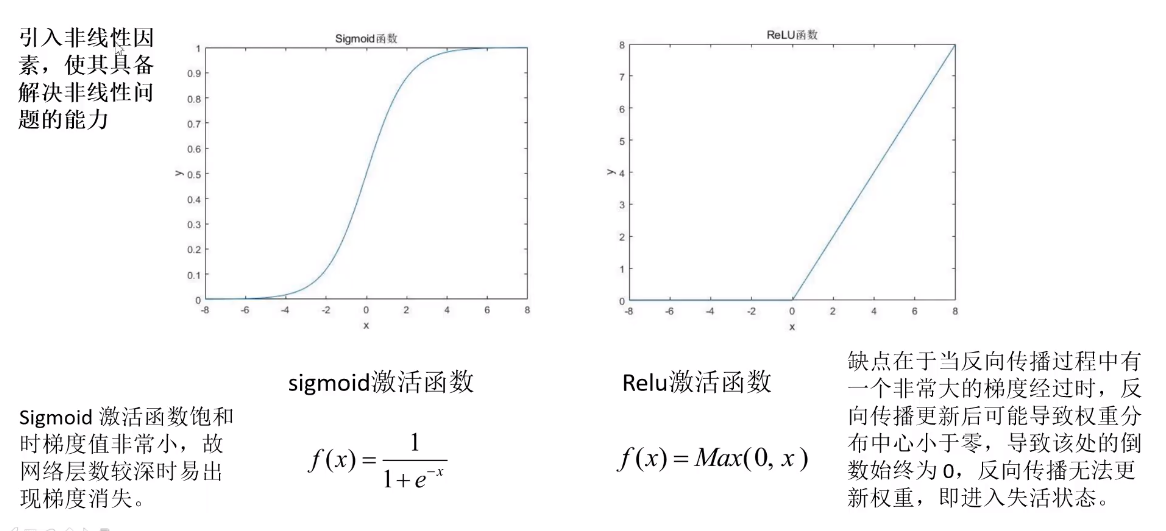鉴于ReLU激活函数的缺点，在开始进行训练的时候，学习率大的话，容易导致激活函数失活。

③  针对卷积过程中出现的越界现象，进行padding处理：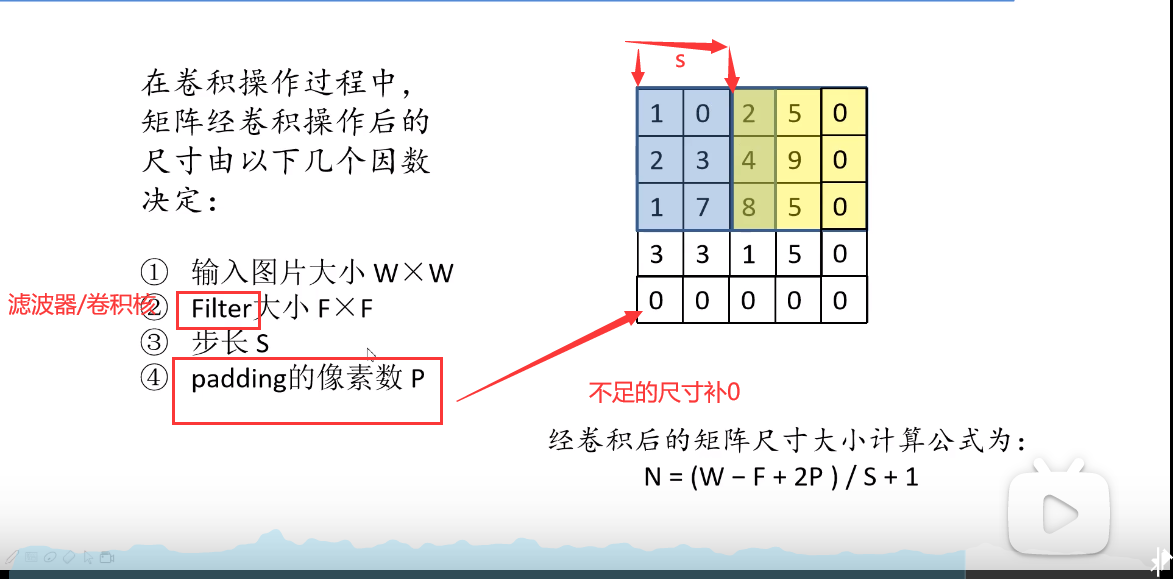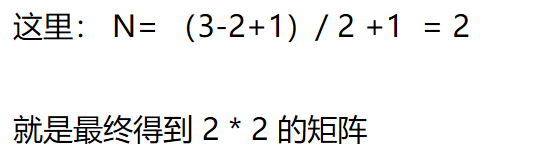3、池化层
① 池化操作方式

池化层的作用：对图像的特征进行稀疏处理，以减少运算量，同时维持图像的特征

下图处理 为：取特征最大值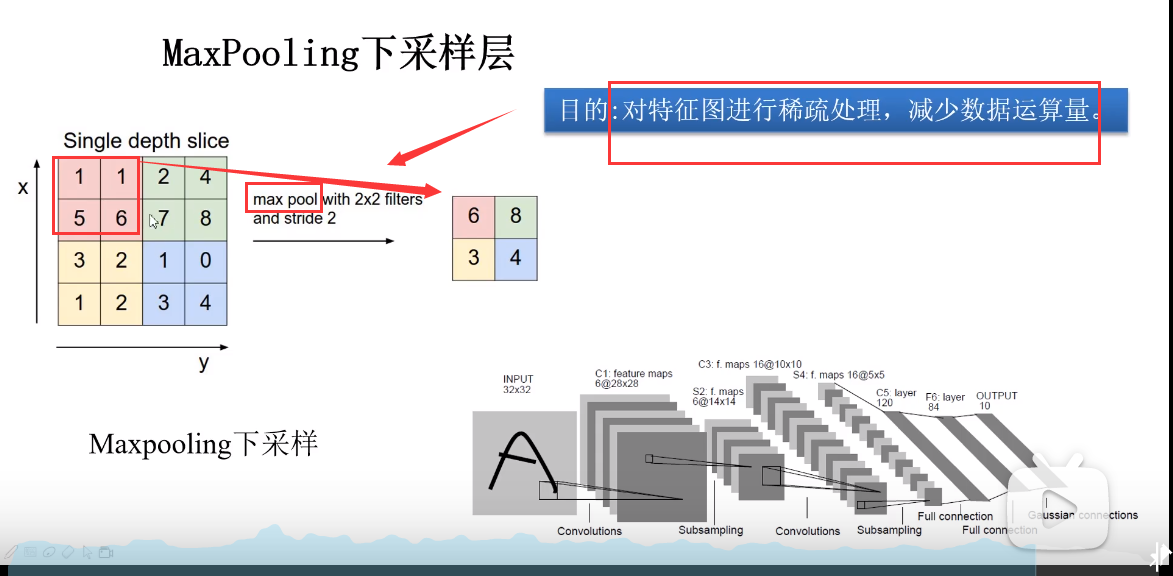下图处理 为：取平均特征值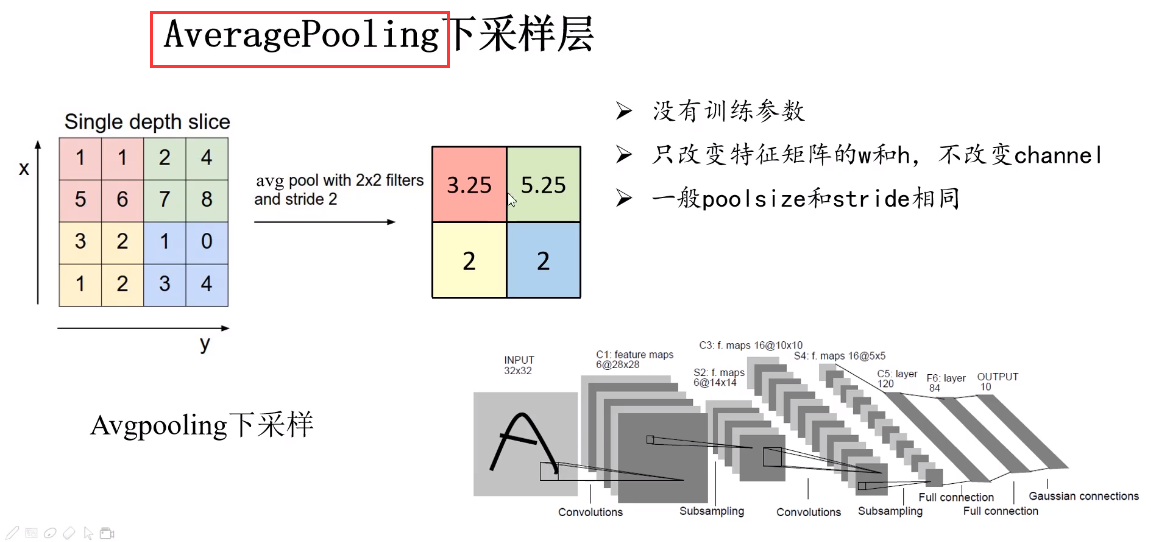② 池化的特点

① 不像卷积（卷积核）一样，有训练参数，池化过程没有训练参数
② 池化操作只改变了特征矩阵的 宽W 和 高h
③ 一般 poolsize 的大小和 stride 步长相同

持续更新ing…

哔站up主视频链接： https://www.bilibili.com/video/BV1M7411M7D2


展开全文深度学习 计算机视觉 人工智能
• 卷积神经网络的具体操作卷积过程代码实现4.池化层(Pooling Sampling)采样上采样5.批处理规范(Batch Norm)Image NormalizationBatch Normalization 1.关于图像基础知识 灰度 这里以MINST数据集为例，对于一个...人工智能
• 基于机器自主识别机场区域检测方法是目前主流检测方法,针对传统检测算法对机场区域遥感图像中多类别、多尺度、多视角以及复杂背景检测鲁棒性不足问题,本文提出了一种优化区域卷积神经网络检测算法。...
• 看到有些答案是刚开始随机初始化卷积核大小，卷积层数和map个数是根据经验来设定，但这个里面应该是有深层次原因吧，比如下面手写字卷积神经网络结构1，最后输出为什么是12个map，即输出12个特征？然后2又是...
• CNN 价值： 能够将大数据量图片有效降维成小数据量(并不影响结果) 能够保留图片特征，类似人类...卷积层运算过程如下图，用一个卷积核扫完整张图片： 池化层（下采样） — — 数据降维，避免过拟合人工智能
• 根据卷积神经网络各个层具有大小不同感受野，从不同层提取多个尺度特征向量分别进行人脸分类与回归，并将网络全连接层改成卷积层，以适应不同大小图片输入。该方法将人脸检测多个步骤集成到一个卷积神经...
• TensorFlow 使用预训练好的卷积神经网络和循环神经网络（lstm）模型处理图片转文字（im2txt） 这是AI大智慧系列文章中的一篇关于图片转文字的一篇博文，介绍了如果使用已经训练好的模型。由于本模型的训练非常耗...
• 卷积神经网络结构简述（二）Inception系列网络WILL深度学习搬砖者​关注他50 人赞了该文章作为一名初入茅庐搬砖者，最近在用深度学习做图像分类任务，粗略学习了下卷积神经网络近几年结构升级。根据个人理.....
• 7-卷积神经网络-读书笔记1. 概述2. 自己动手搭CNN3. 经典CNN结构探索4. 课程实践 ...1.2.1 卷积神经网络的诞生 卷积：平移不变模式 池化：采样被检测物体不变模式 1.2.2 CNN基础结构 CNN应用...算法
• 也就是基于FPGA的卷积神经网络实现里面的top.v文件。 本项目要实现的神经网络结构如下图所示： 对于在FPGA当中实现神经网络而言，每一种功能的层都单独设计一个子模块。而使用这些子模块搭建一个完整的网络框架主要...fpga
• 提出了一种基于显著性和深度卷积神经网络的输送带表面故障定位方法。该方法在输送带上、表面边缘烙上数字,利用图像处理技术检测输送带图像中数字来间接定位输送带表面故障。首先,将采集输送带图像进行高斯...
• 看到有些答案是刚开始随机初始化卷积核大小，卷积层数和map个数是根据经验来设定，但这个里面应该是有深层次原因吧，比如下面手写字卷积神经网络结构1，最后输出为什么是12个map，即输出12个特征？然后2又是...深度学习 人工智能
• 利用DCGAN-生成对抗网络和CNN-卷积神经网络对图像进行训练和分类 总体流程步骤 一、图像处理部分 如下图，分别是由SAR卫星获取极化SAR图像及其标签图 根据不同颜色标签（红、绿、黄、白）可以将原图分为四个...python 深度学习 程序人生
• 神经网络由输入层、隐藏层和输出层组成，长相如下图所示。对于一些问题来说用线性回归可以解决，但有些问题用线性很麻烦，而用神经网络就很轻松了，各有各优缺点。对于神经网络来说，是一种非线性，输入参数，...
• TensorFlow 使用预训练好的卷积神经网络和循环神经网络（lstm）模型处理图片转文字（im2txt） 这是AI大智慧系列文章中的一篇关于图片转文字的一篇博文，介绍了如果使用已经训练好的模型。由于本模型的训练非常耗...
• 该存储库包含一些笔记本，用于学习在给定一组训练数据情况如何使用卷积神经网络（CNN）创建图像分类器。 这些笔记本包括用于学习构成卷积神经网络的各个图层示例，以及在数据集上训练示例服装分类器。 设置......